Скачать презентацию DECISION MODELING WITH MICROSOFT EXCEL Chapter 9 Monte

d46b10f58bbdded2fcce7322d9e137d6.ppt

• Количество слайдов: 76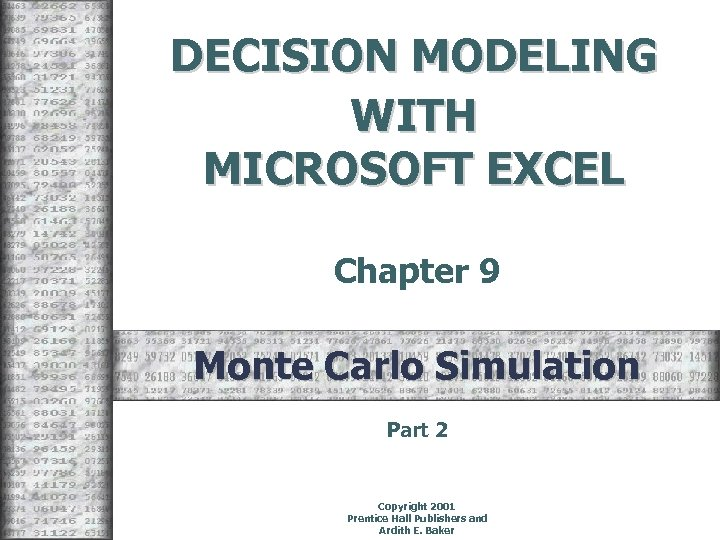DECISION MODELING WITH MICROSOFT EXCEL Chapter 9 Monte Carlo Simulation Part 2 Copyright 2001 Prentice Hall Publishers and Ardith E. Baker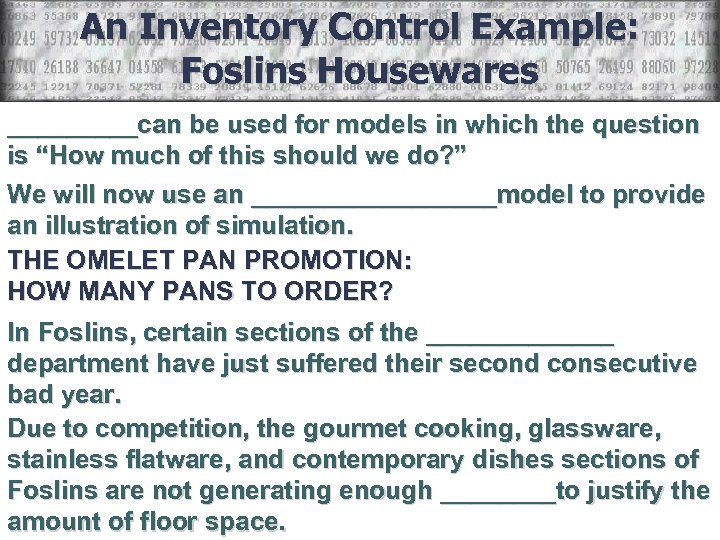An Inventory Control Example: Foslins Housewares _____can be used for models in which the question is “How much of this should we do? ” We will now use an _________model to provide an illustration of simulation. THE OMELET PAN PROMOTION: HOW MANY PANS TO ORDER? In Foslins, certain sections of the _______ department have just suffered their second consecutive bad year. Due to competition, the gourmet cooking, glassware, stainless flatware, and contemporary dishes sections of Foslins are not generating enough ____to justify the amount of floor space.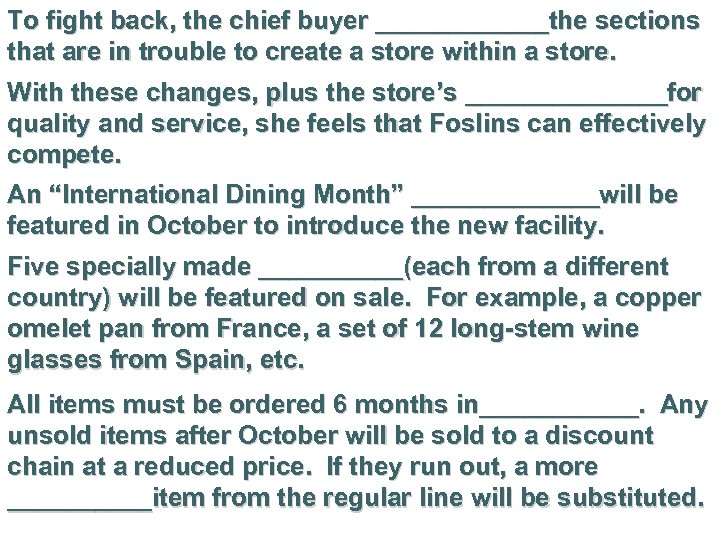To fight back, the chief buyer ______the sections that are in trouble to create a store within a store. With these changes, plus the store’s _______for quality and service, she feels that Foslins can effectively compete. An “International Dining Month” _______will be featured in October to introduce the new facility. Five specially made _____(each from a different country) will be featured on sale. For example, a copper omelet pan from France, a set of 12 long-stem wine glasses from Spain, etc. All items must be ordered 6 months in______. Any unsold items after October will be sold to a discount chain at a reduced price. If they run out, a more _____item from the regular line will be substituted.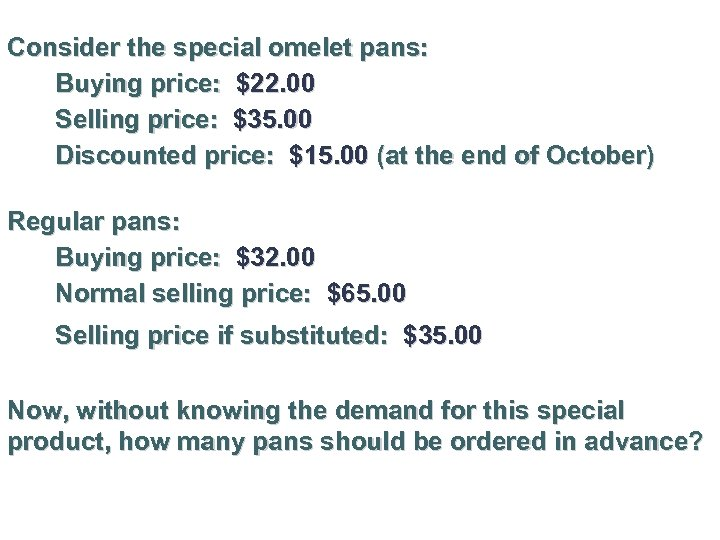Consider the special omelet pans: Buying price: \$22. 00 Selling price: \$35. 00 Discounted price: \$15. 00 (at the end of October) Regular pans: Buying price: \$32. 00 Normal selling price: \$65. 00 Selling price if substituted: \$35. 00 Now, without knowing the demand for this special product, how many pans should be ordered in advance?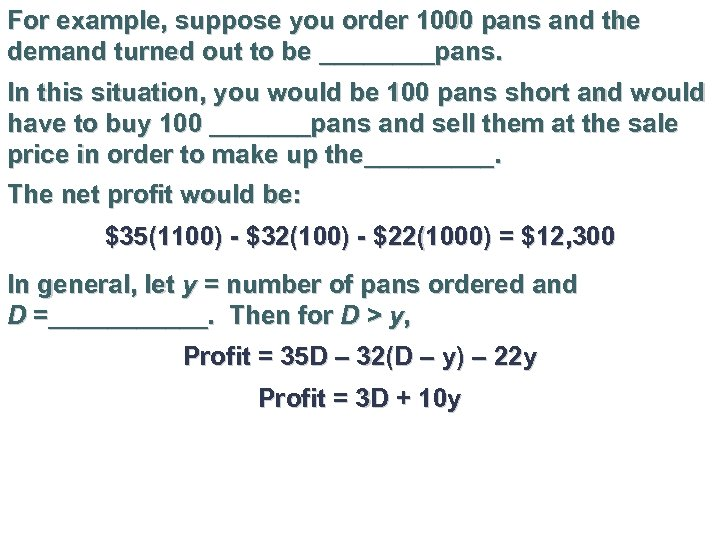For example, suppose you order 1000 pans and the demand turned out to be ____pans. In this situation, you would be 100 pans short and would have to buy 100 _______pans and sell them at the sale price in order to make up the_____. The net profit would be: \$35(1100) - \$32(100) - \$22(1000) = \$12, 300 In general, let y = number of pans ordered and D =______. Then for D > y, Profit = 35 D – 32(D – y) – 22 y Profit = 3 D + 10 y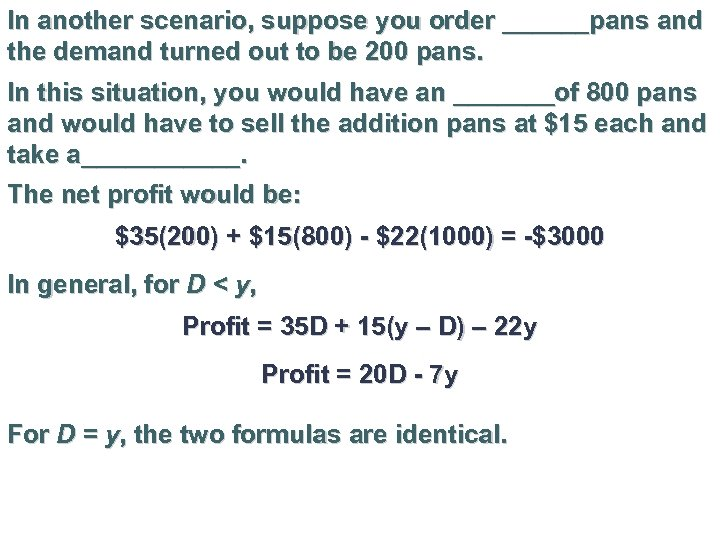In another scenario, suppose you order ______pans and the demand turned out to be 200 pans. In this situation, you would have an _______of 800 pans and would have to sell the addition pans at \$15 each and take a______. The net profit would be: \$35(200) + \$15(800) - \$22(1000) = -\$3000 In general, for D < y, Profit = 35 D + 15(y – D) – 22 y Profit = 20 D - 7 y For D = y, the two formulas are identical.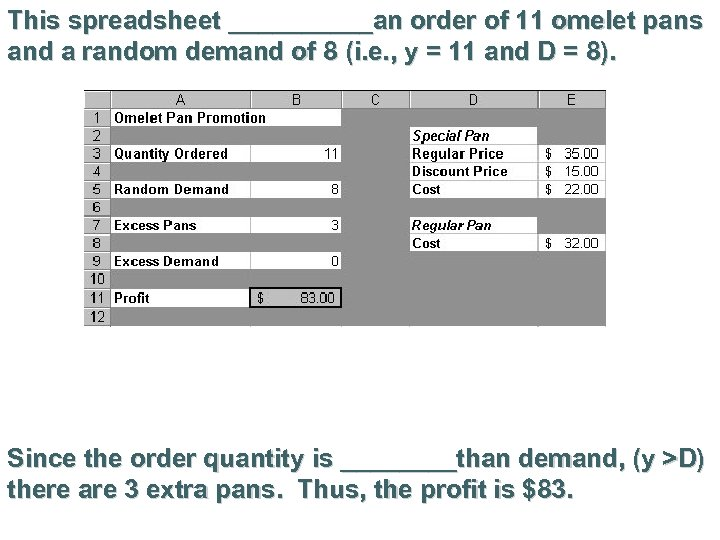This spreadsheet _____an order of 11 omelet pans and a random demand of 8 (i. e. , y = 11 and D = 8). Since the order quantity is ____than demand, (y >D) there are 3 extra pans. Thus, the profit is \$83.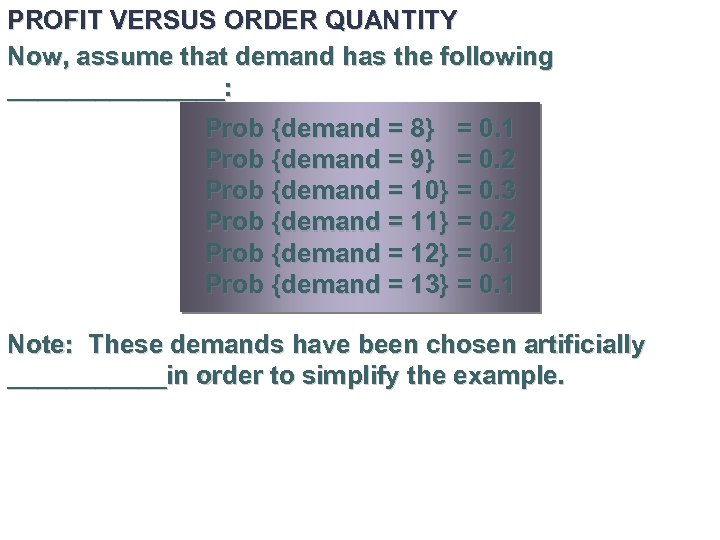PROFIT VERSUS ORDER QUANTITY Now, assume that demand has the following ________: Prob {demand = 8} = 0. 1 Prob {demand = 9} = 0. 2 Prob {demand = 10} = 0. 3 Prob {demand = 11} = 0. 2 Prob {demand = 12} = 0. 1 Prob {demand = 13} = 0. 1 Note: These demands have been chosen artificially ______in order to simplify the example.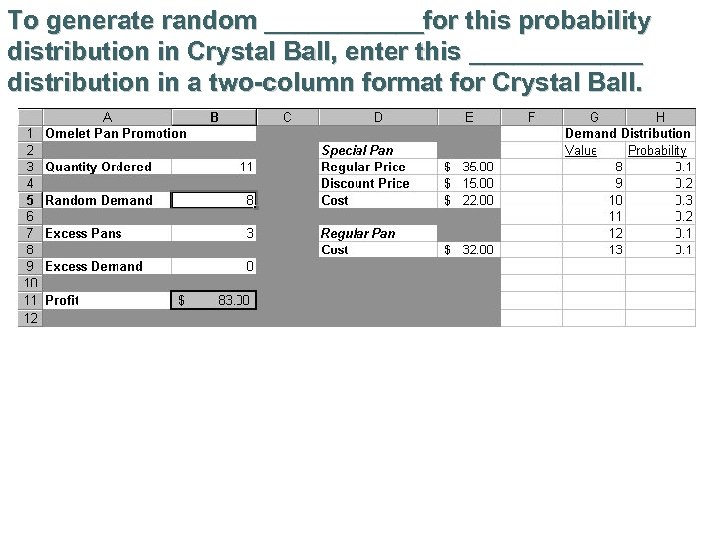To generate random ______for this probability distribution in Crystal Ball, enter this ______ distribution in a two-column format for Crystal Ball.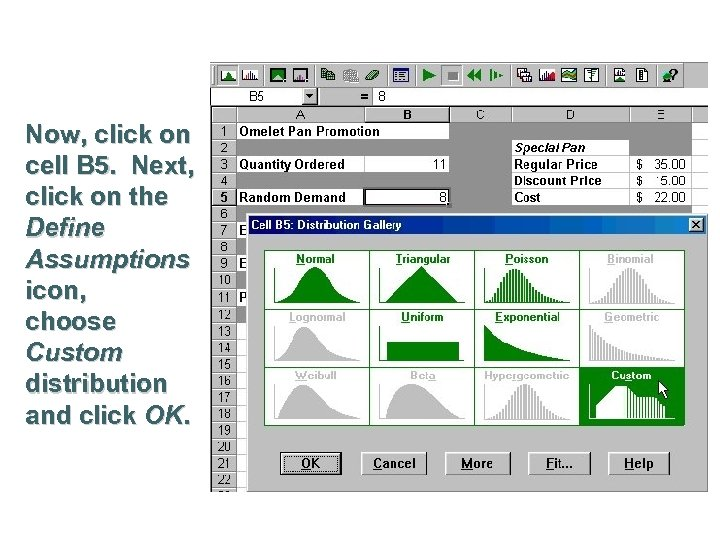Now, click on cell B 5. Next, click on the Define Assumptions icon, choose Custom distribution and click OK.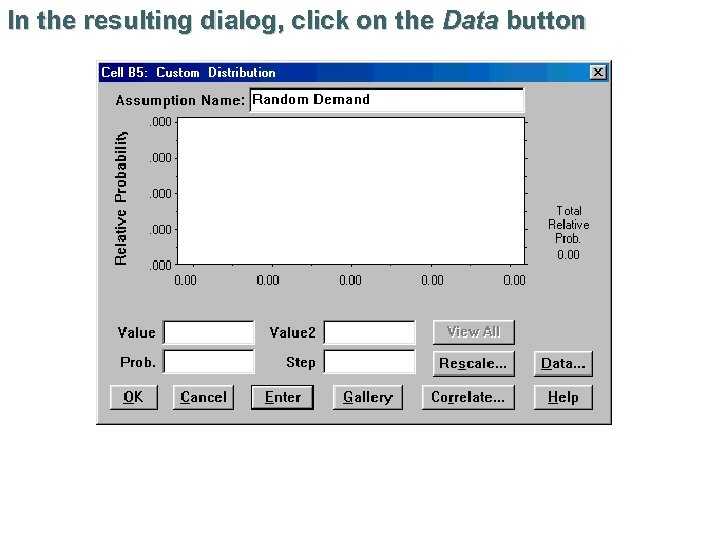In the resulting dialog, click on the Data button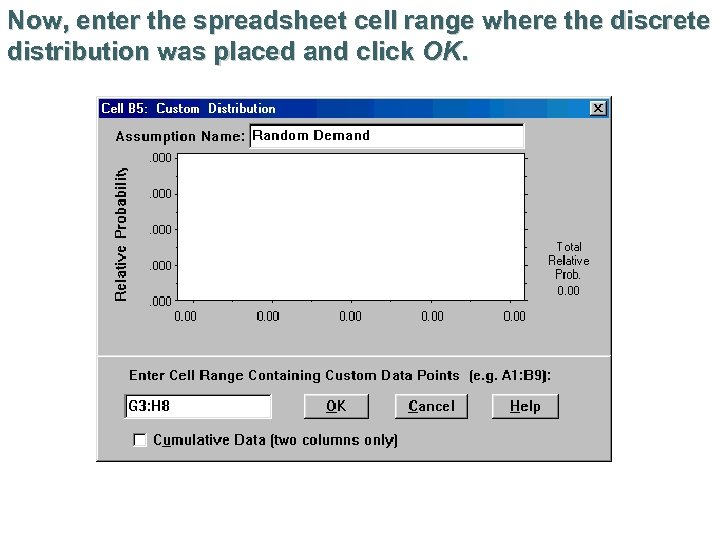Now, enter the spreadsheet cell range where the discrete distribution was placed and click OK.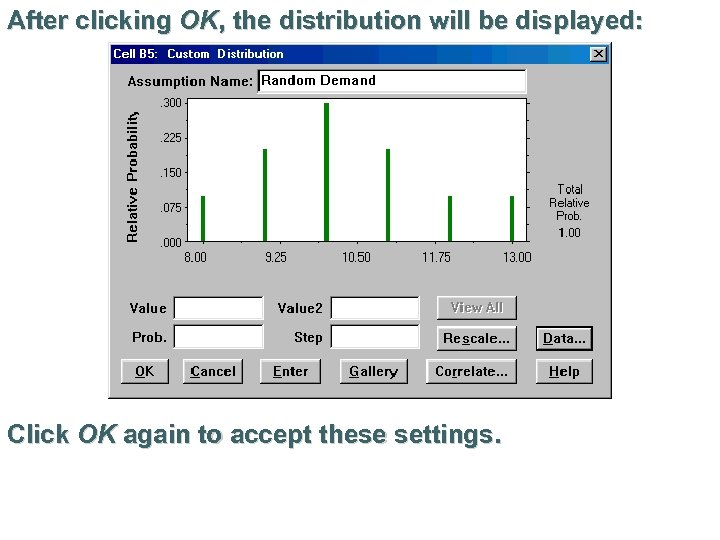After clicking OK, the distribution will be displayed: Click OK again to accept these settings.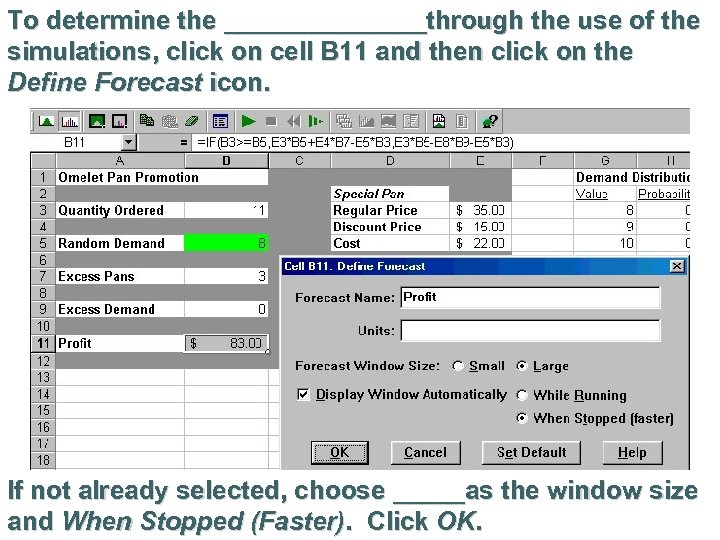To determine the _______through the use of the simulations, click on cell B 11 and then click on the Define Forecast icon. If not already selected, choose _____as the window size and When Stopped (Faster). Click OK.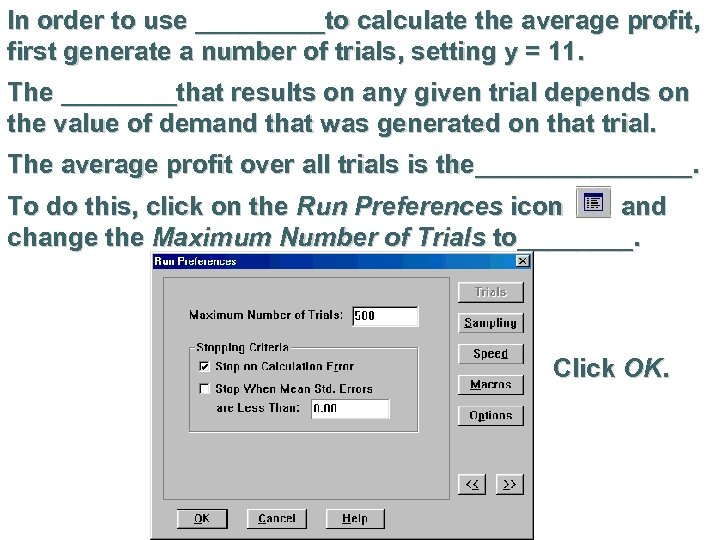In order to use _____to calculate the average profit, first generate a number of trials, setting y = 11. The ____that results on any given trial depends on the value of demand that was generated on that trial. The average profit over all trials is the________. To do this, click on the Run Preferences icon and change the Maximum Number of Trials to____. Click OK.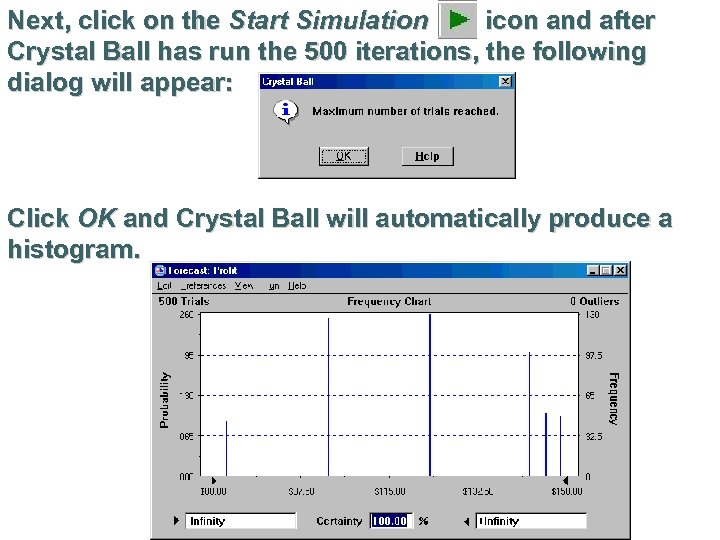Next, click on the Start Simulation icon and after Crystal Ball has run the 500 iterations, the following dialog will appear: Click OK and Crystal Ball will automatically produce a histogram.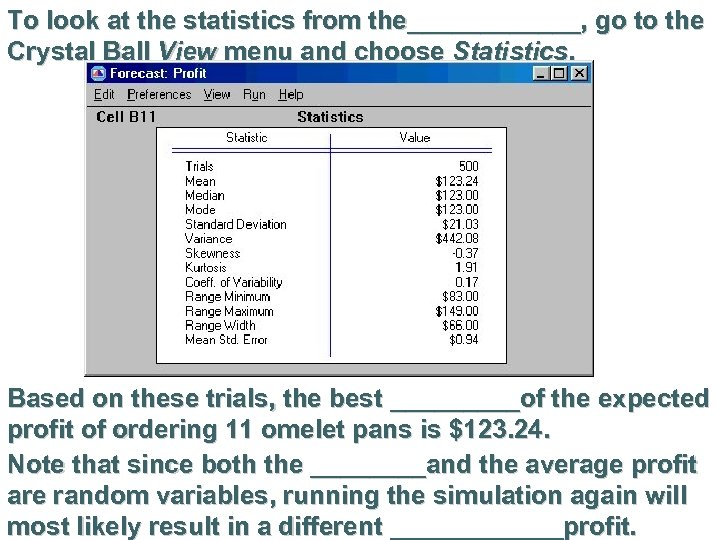To look at the statistics from the______, go to the Crystal Ball View menu and choose Statistics. Based on these trials, the best _____of the expected profit of ordering 11 omelet pans is \$123. 24. Note that since both the ____and the average profit are random variables, running the simulation again will most likely result in a different ______profit.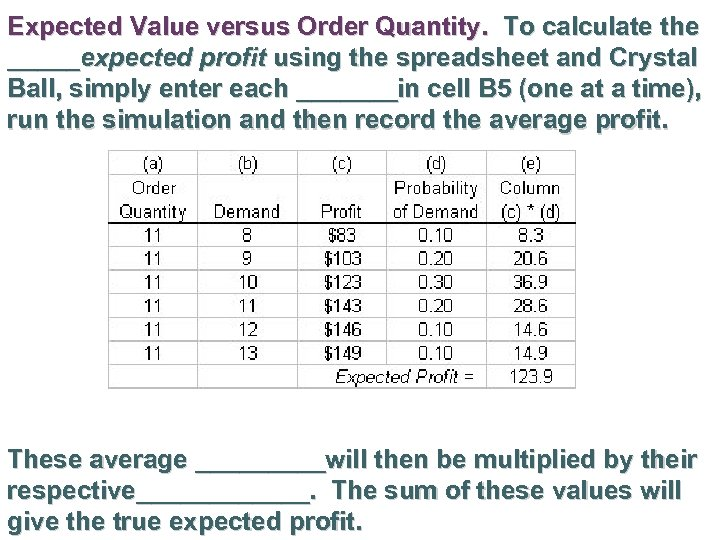Expected Value versus Order Quantity. To calculate the _____expected profit using the spreadsheet and Crystal Ball, simply enter each _______in cell B 5 (one at a time), run the simulation and then record the average profit. These average _____will then be multiplied by their respective______. The sum of these values will give the true expected profit.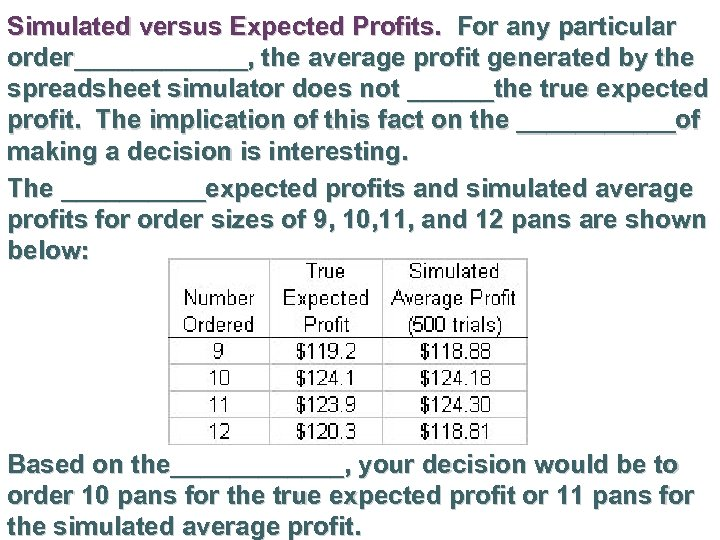Simulated versus Expected Profits. For any particular order______, the average profit generated by the spreadsheet simulator does not ______the true expected profit. The implication of this fact on the ______of making a decision is interesting. The _____expected profits and simulated average profits for order sizes of 9, 10, 11, and 12 pans are shown below: Based on the______, your decision would be to order 10 pans for the true expected profit or 11 pans for the simulated average profit.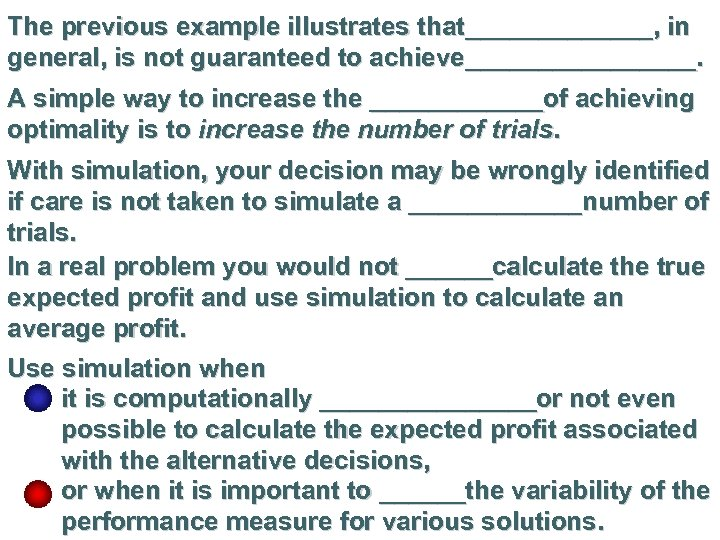The previous example illustrates that_______, in general, is not guaranteed to achieve________. A simple way to increase the ______of achieving optimality is to increase the number of trials. With simulation, your decision may be wrongly identified if care is not taken to simulate a ______number of trials. In a real problem you would not ______calculate the true expected profit and use simulation to calculate an average profit. Use simulation when it is computationally ________or not even possible to calculate the expected profit associated with the alternative decisions, or when it is important to ______the variability of the performance measure for various solutions.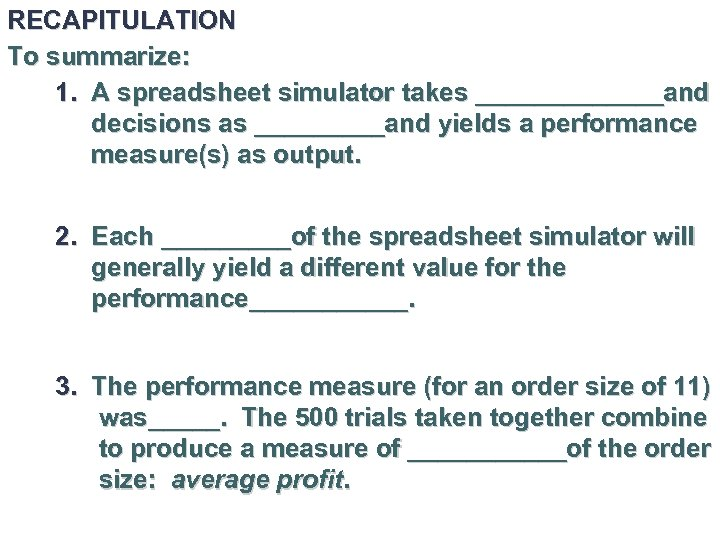RECAPITULATION To summarize: 1. A spreadsheet simulator takes _______and decisions as _____and yields a performance measure(s) as output. 2. Each _____of the spreadsheet simulator will generally yield a different value for the performance______. 3. The performance measure (for an order size of 11) was_____. The 500 trials taken together combine to produce a measure of ______of the order size: average profit.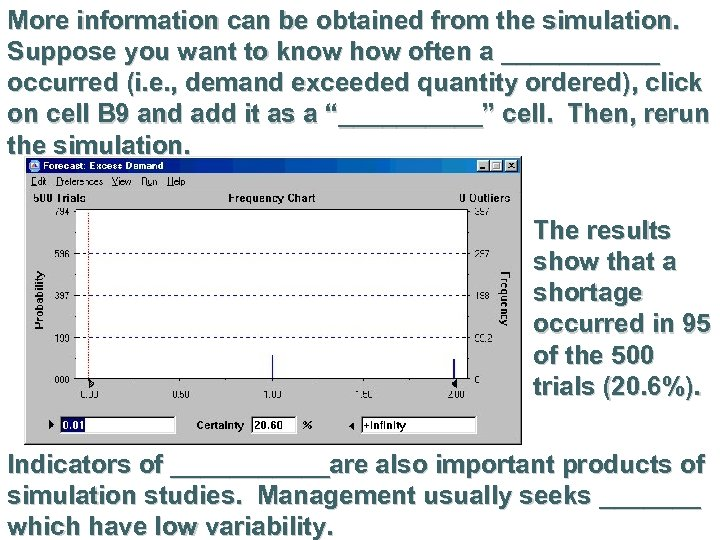More information can be obtained from the simulation. Suppose you want to know how often a ______ occurred (i. e. , demand exceeded quantity ordered), click on cell B 9 and add it as a “_____” cell. Then, rerun the simulation. The results show that a shortage occurred in 95 of the 500 trials (20. 6%). Indicators of ______are also important products of simulation studies. Management usually seeks _______ which have low variability.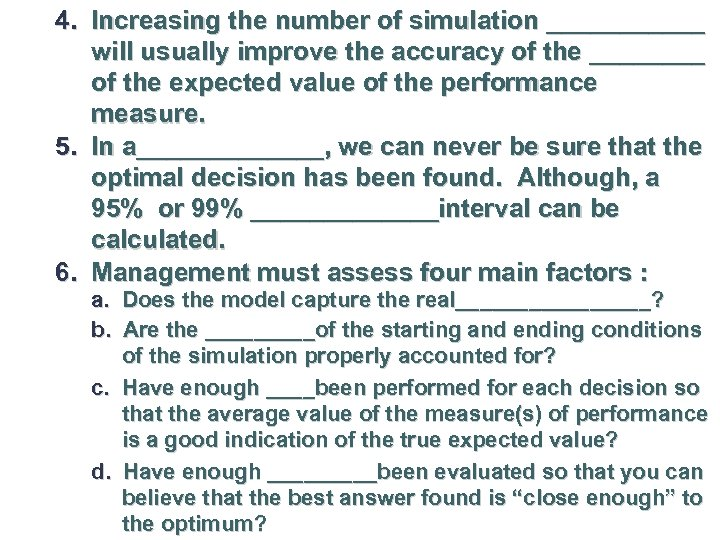4. Increasing the number of simulation ______ will usually improve the accuracy of the ____ of the expected value of the performance measure. 5. In a_______, we can never be sure that the optimal decision has been found. Although, a 95% or 99% _______interval can be calculated. 6. Management must assess four main factors : a. Does the model capture the real________? b. Are the _____of the starting and ending conditions of the simulation properly accounted for? c. Have enough ____been performed for each decision so that the average value of the measure(s) of performance is a good indication of the true expected value? d. Have enough _____been evaluated so that you can believe that the best answer found is “close enough” to the optimum?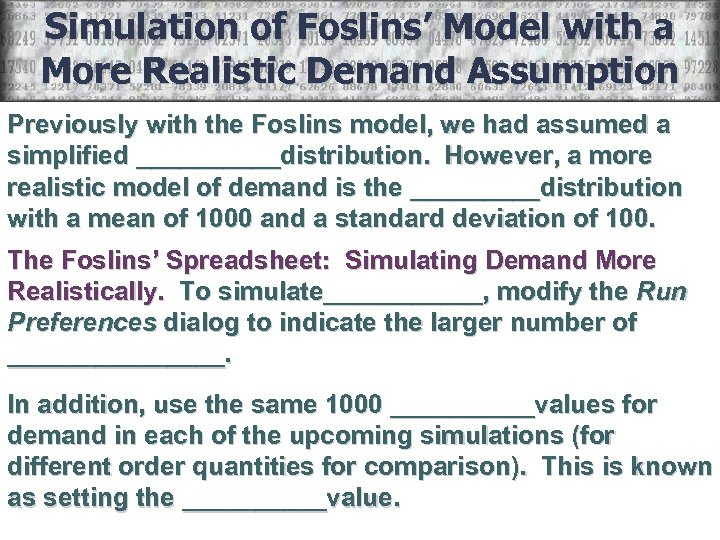Simulation of Foslins’ Model with a More Realistic Demand Assumption Previously with the Foslins model, we had assumed a simplified _____distribution. However, a more realistic model of demand is the _____distribution with a mean of 1000 and a standard deviation of 100. The Foslins’ Spreadsheet: Simulating Demand More Realistically. To simulate______, modify the Run Preferences dialog to indicate the larger number of ________. In addition, use the same 1000 _____values for demand in each of the upcoming simulations (for different order quantities for comparison). This is known as setting the _____value.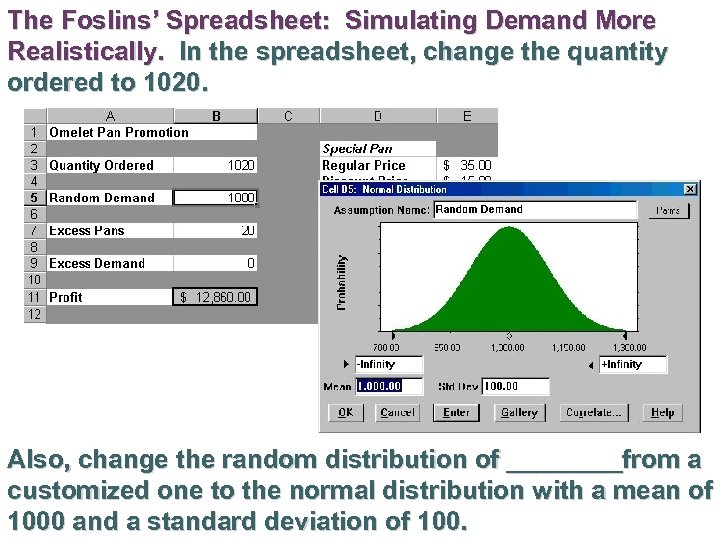The Foslins’ Spreadsheet: Simulating Demand More Realistically. In the spreadsheet, change the quantity ordered to 1020. Also, change the random distribution of ____from a customized one to the normal distribution with a mean of 1000 and a standard deviation of 100.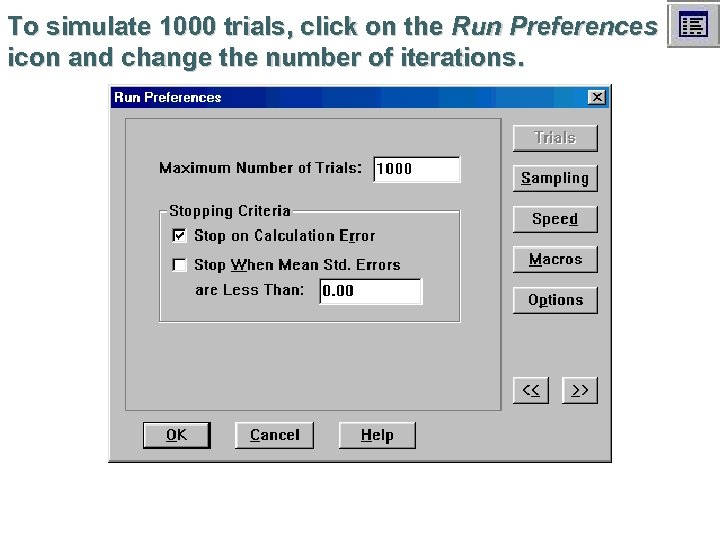To simulate 1000 trials, click on the Run Preferences icon and change the number of iterations.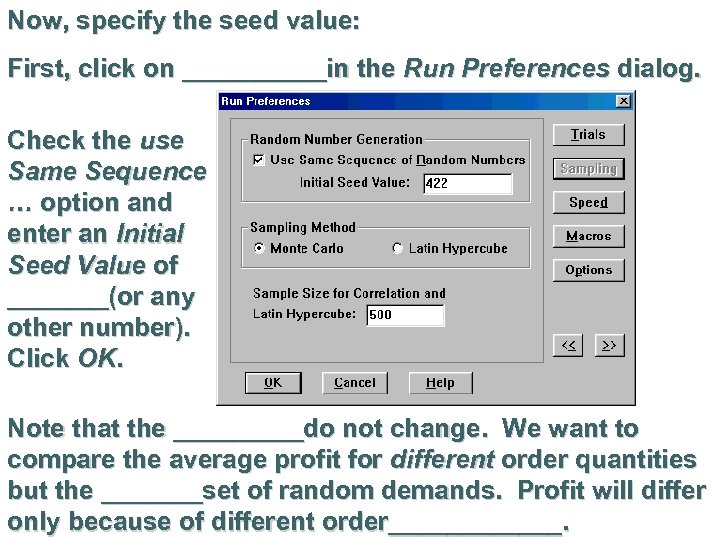Now, specify the seed value: First, click on _____in the Run Preferences dialog. Check the use Same Sequence … option and enter an Initial Seed Value of _______(or any other number). Click OK. Note that the _____do not change. We want to compare the average profit for different order quantities but the _______set of random demands. Profit will differ only because of different order______.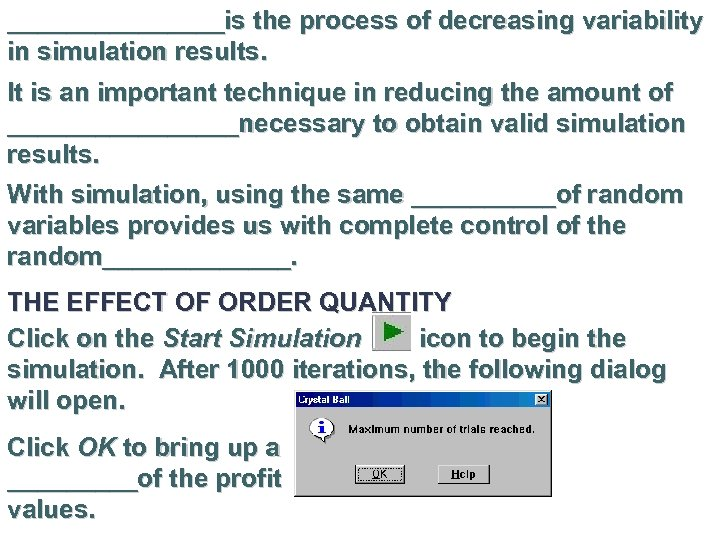________is the process of decreasing variability in simulation results. It is an important technique in reducing the amount of ________necessary to obtain valid simulation results. With simulation, using the same _____of random variables provides us with complete control of the random_______. THE EFFECT OF ORDER QUANTITY Click on the Start Simulation icon to begin the simulation. After 1000 iterations, the following dialog will open. Click OK to bring up a _____of the profit values.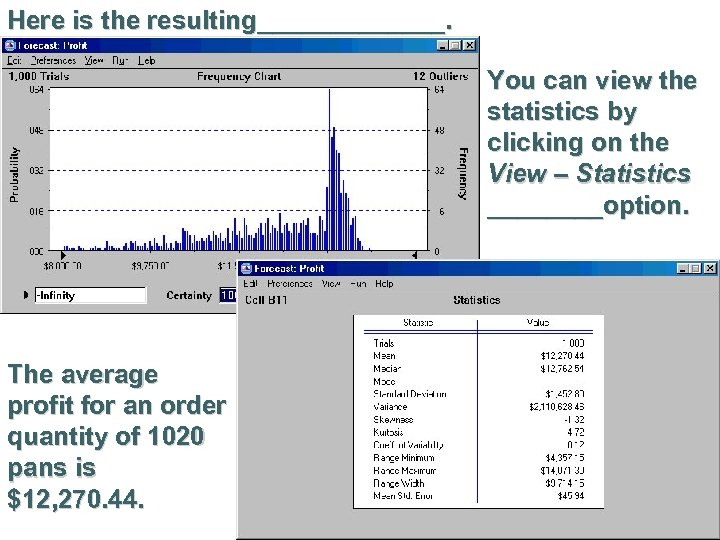Here is the resulting_______. You can view the statistics by clicking on the View – Statistics ____option. The average profit for an order quantity of 1020 pans is \$12, 270. 44.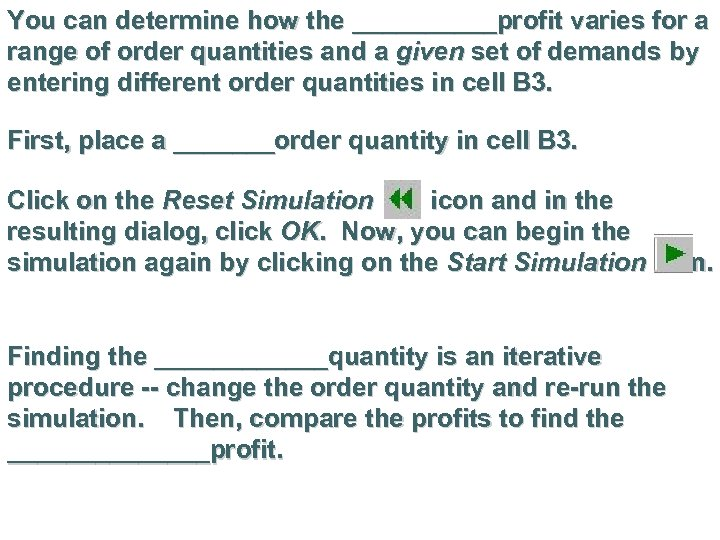You can determine how the _____profit varies for a range of order quantities and a given set of demands by entering different order quantities in cell B 3. First, place a _______order quantity in cell B 3. Click on the Reset Simulation icon and in the resulting dialog, click OK. Now, you can begin the simulation again by clicking on the Start Simulation icon. Finding the ______quantity is an iterative procedure -- change the order quantity and re-run the simulation. Then, compare the profits to find the _______profit.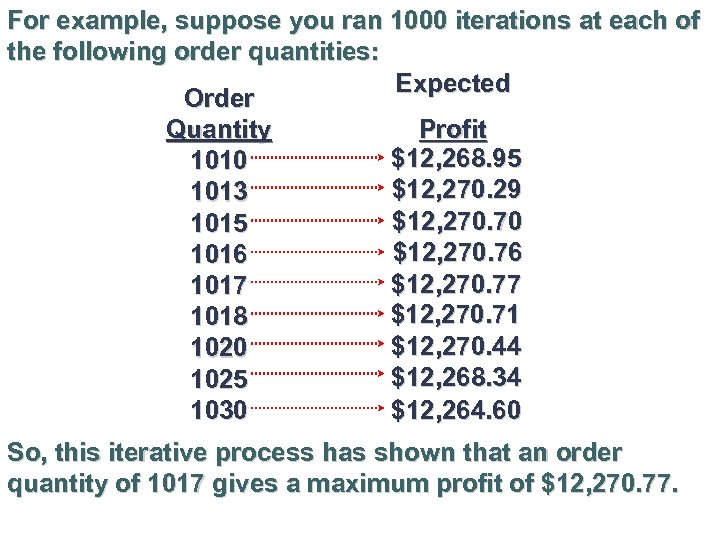For example, suppose you ran 1000 iterations at each of the following order quantities: Expected Order Quantity Profit \$12, 268. 95 1010 \$12, 270. 29 1013 \$12, 270. 70 1015 \$12, 270. 76 1016 \$12, 270. 77 1017 \$12, 270. 71 1018 \$12, 270. 44 1020 \$12, 268. 34 1025 \$12, 264. 60 1030 So, this iterative process has shown that an order quantity of 1017 gives a maximum profit of \$12, 270. 77.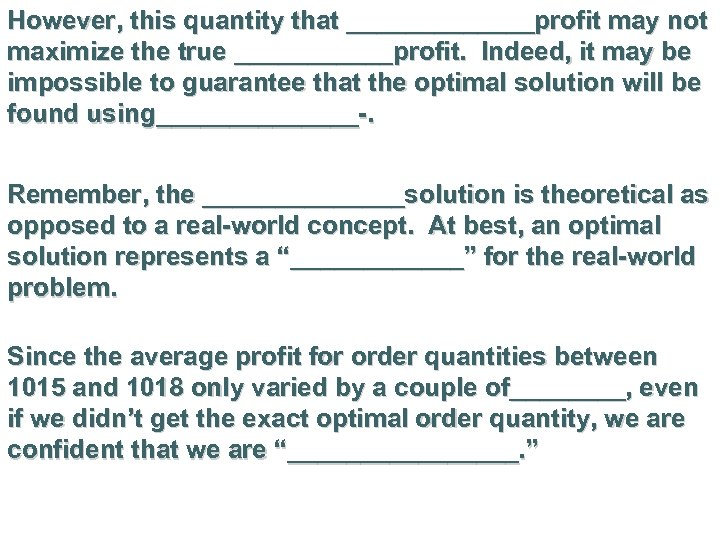However, this quantity that _______profit may not maximize the true ______profit. Indeed, it may be impossible to guarantee that the optimal solution will be found using_______-. Remember, the _______solution is theoretical as opposed to a real-world concept. At best, an optimal solution represents a “______” for the real-world problem. Since the average profit for order quantities between 1015 and 1018 only varied by a couple of____, even if we didn’t get the exact optimal order quantity, we are confident that we are “________. ”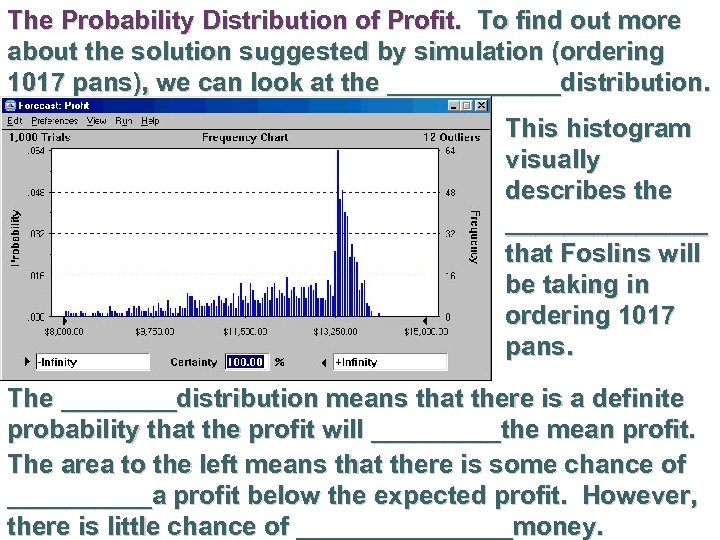The Probability Distribution of Profit. To find out more about the solution suggested by simulation (ordering 1017 pans), we can look at the ______distribution. This histogram visually describes the _______ that Foslins will be taking in ordering 1017 pans. The ____distribution means that there is a definite probability that the profit will _____the mean profit. The area to the left means that there is some chance of _____a profit below the expected profit. However, there is little chance of ________money.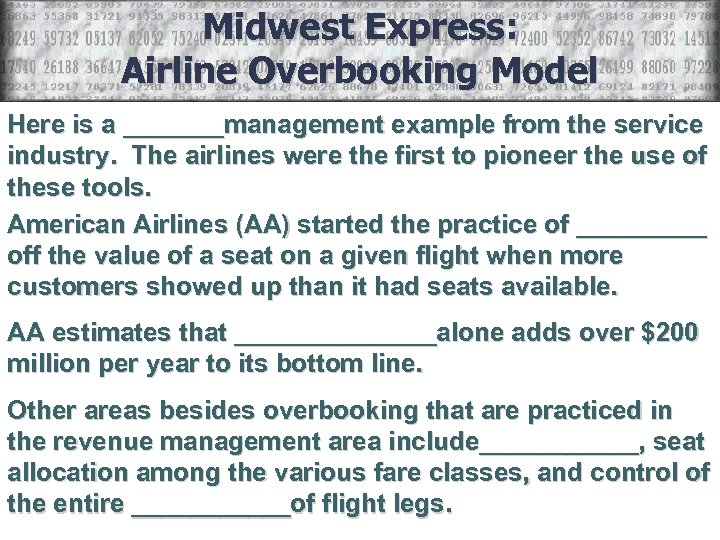Midwest Express: Airline Overbooking Model Here is a _______management example from the service industry. The airlines were the first to pioneer the use of these tools. American Airlines (AA) started the practice of _____ off the value of a seat on a given flight when more customers showed up than it had seats available. AA estimates that _______alone adds over \$200 million per year to its bottom line. Other areas besides overbooking that are practiced in the revenue management area include______, seat allocation among the various fare classes, and control of the entire ______of flight legs.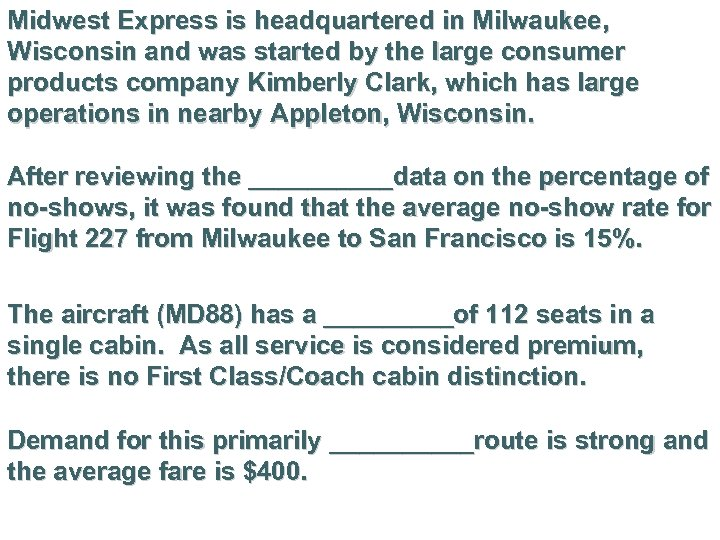Midwest Express is headquartered in Milwaukee, Wisconsin and was started by the large consumer products company Kimberly Clark, which has large operations in nearby Appleton, Wisconsin. After reviewing the _____data on the percentage of no-shows, it was found that the average no-show rate for Flight 227 from Milwaukee to San Francisco is 15%. The aircraft (MD 88) has a _____of 112 seats in a single cabin. As all service is considered premium, there is no First Class/Coach cabin distinction. Demand for this primarily _____route is strong and the average fare is \$400.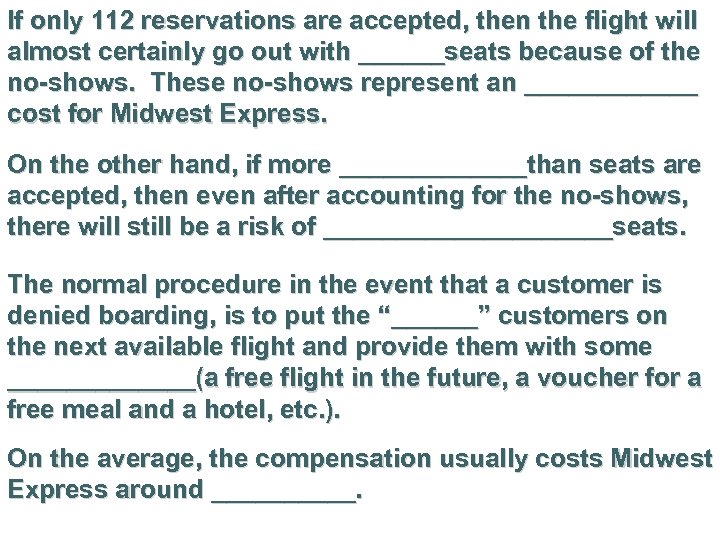If only 112 reservations are accepted, then the flight will almost certainly go out with ______seats because of the no-shows. These no-shows represent an ______ cost for Midwest Express. On the other hand, if more _______than seats are accepted, then even after accounting for the no-shows, there will still be a risk of __________seats. The normal procedure in the event that a customer is denied boarding, is to put the “______” customers on the next available flight and provide them with some _______(a free flight in the future, a voucher for a free meal and a hotel, etc. ). On the average, the compensation usually costs Midwest Express around _____.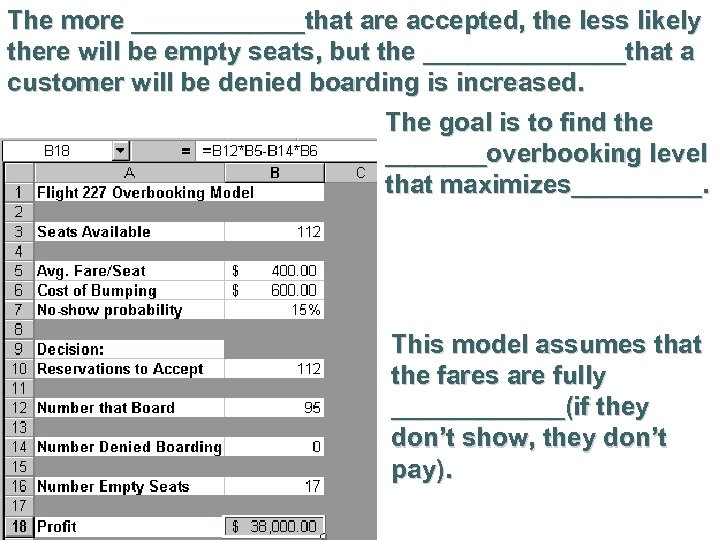The more ______that are accepted, the less likely there will be empty seats, but the _______that a customer will be denied boarding is increased. The goal is to find the _______overbooking level that maximizes_____. This model assumes that the fares are fully ______(if they don’t show, they don’t pay).You can perform multiple trials using ____since the value returned will change every time the spreadsheet is recalculated by pressing _____. In order to see the _____values returned for each iteration, set @Risk to Monte Carlo by clicking on the Change @Risk Settings icon and then on the Sampling tab. In the Standard Recalc section, click on Monte Carlo.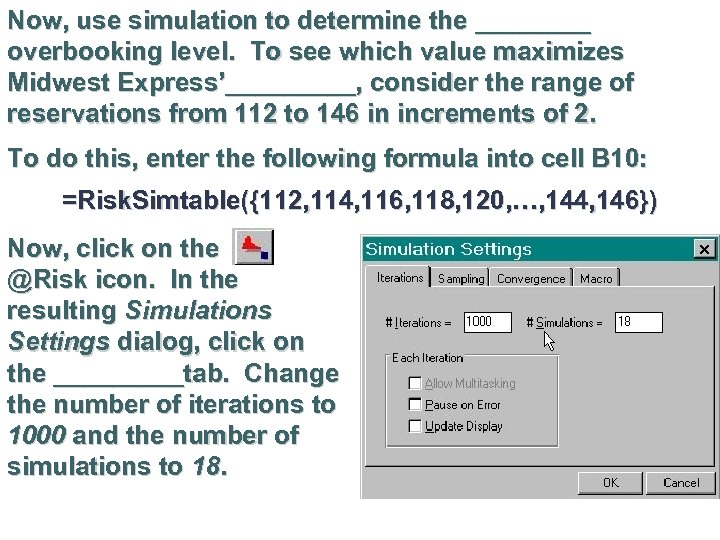Now, use simulation to determine the ____ overbooking level. To see which value maximizes Midwest Express’_____, consider the range of reservations from 112 to 146 in increments of 2. To do this, enter the following formula into cell B 10: =Risk. Simtable({112, 114, 116, 118, 120, …, 144, 146}) Now, click on the @Risk icon. In the resulting Simulations Settings dialog, click on the _____tab. Change the number of iterations to 1000 and the number of simulations to 18.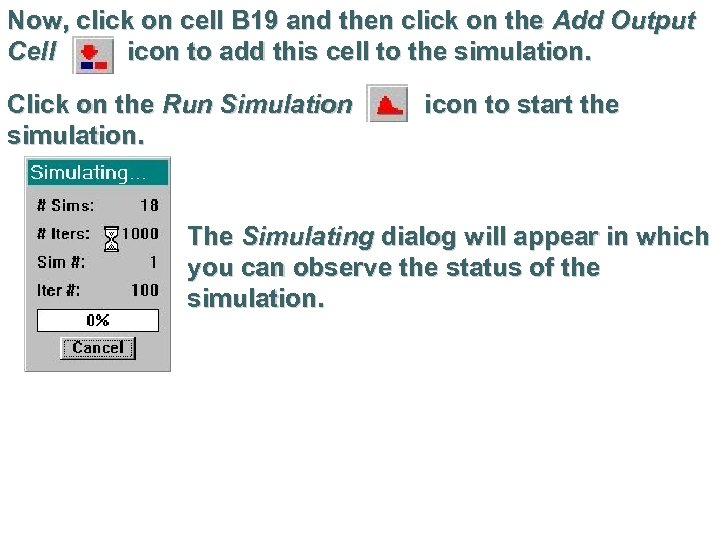Now, click on cell B 19 and then click on the Add Output Cell icon to add this cell to the simulation. Click on the Run Simulation simulation. icon to start the The Simulating dialog will appear in which you can observe the status of the simulation.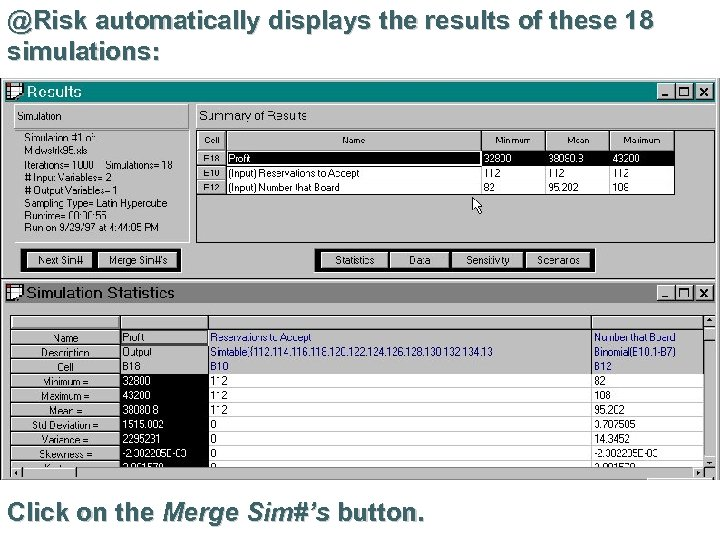@Risk automatically displays the results of these 18 simulations: Click on the Merge Sim#’s button.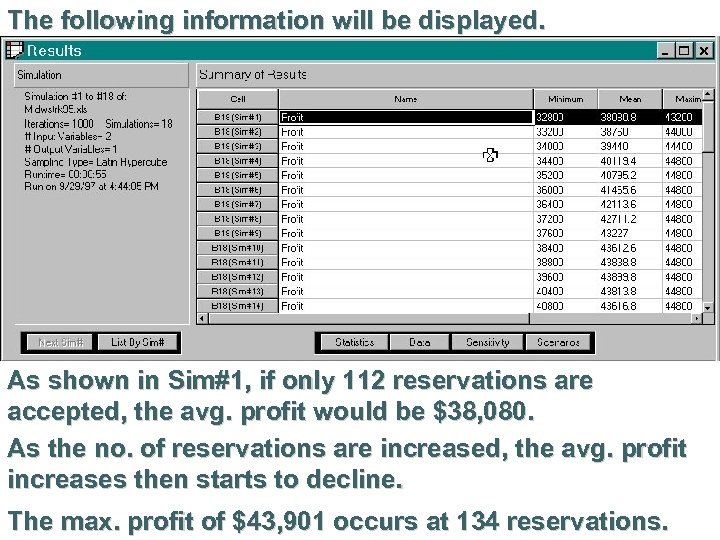The following information will be displayed. As shown in Sim#1, if only 112 reservations are accepted, the avg. profit would be \$38, 080. As the no. of reservations are increased, the avg. profit increases then starts to decline. The max. profit of \$43, 901 occurs at 134 reservations.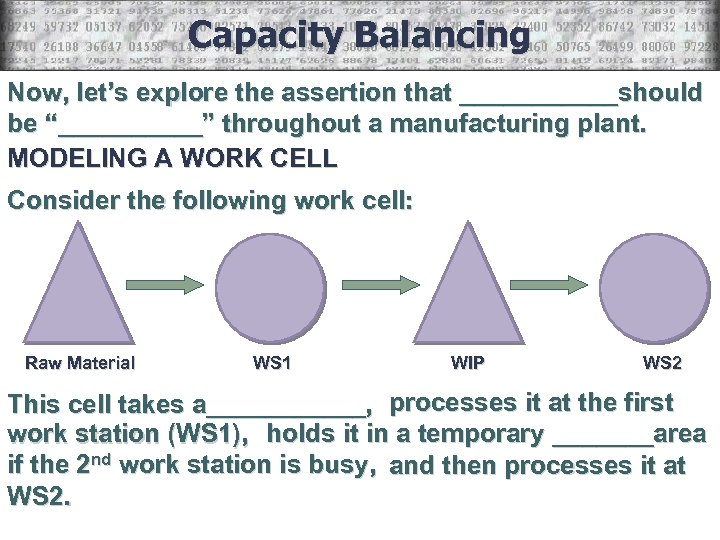Capacity Balancing Now, let’s explore the assertion that ______should be “_____” throughout a manufacturing plant. MODELING A WORK CELL Consider the following work cell: Raw Material WS 1 WIP WS 2 This cell takes a______, processes it at the first work station (WS 1), holds it in a temporary _______area if the 2 nd work station is busy, and then processes it at WS 2.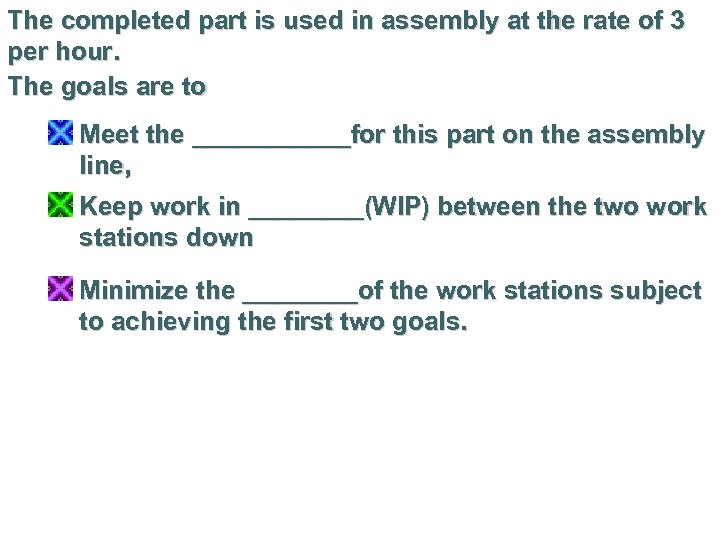The completed part is used in assembly at the rate of 3 per hour. The goals are to Meet the ______for this part on the assembly line, Keep work in ____(WIP) between the two work stations down Minimize the ____of the work stations subject to achieving the first two goals.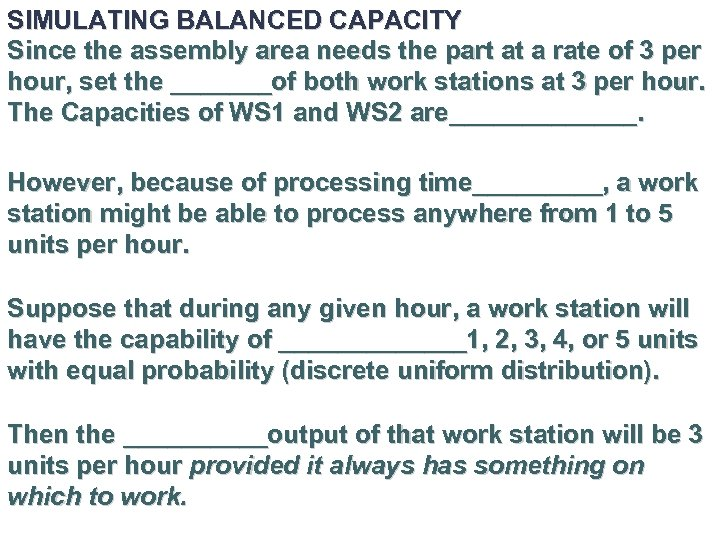SIMULATING BALANCED CAPACITY Since the assembly area needs the part at a rate of 3 per hour, set the _______of both work stations at 3 per hour. The Capacities of WS 1 and WS 2 are_______. However, because of processing time_____, a work station might be able to process anywhere from 1 to 5 units per hour. Suppose that during any given hour, a work station will have the capability of _______1, 2, 3, 4, or 5 units with equal probability (discrete uniform distribution). Then the _____output of that work station will be 3 units per hour provided it always has something on which to work.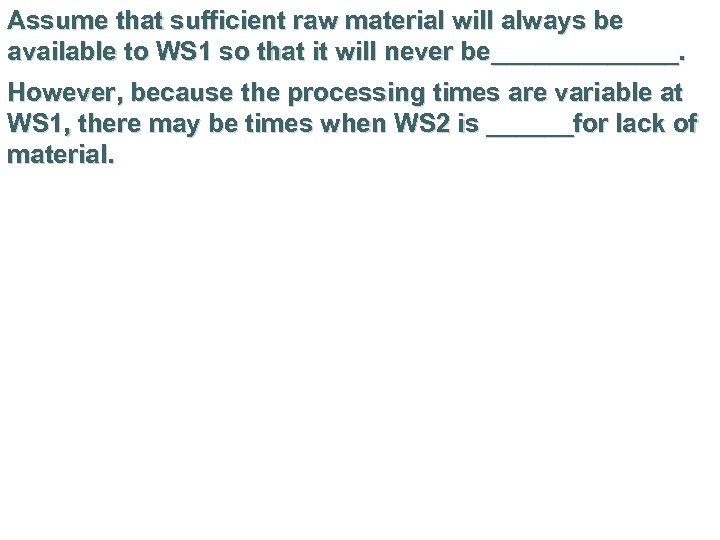Assume that sufficient raw material will always be available to WS 1 so that it will never be_______. However, because the processing times are variable at WS 1, there may be times when WS 2 is ______for lack of material.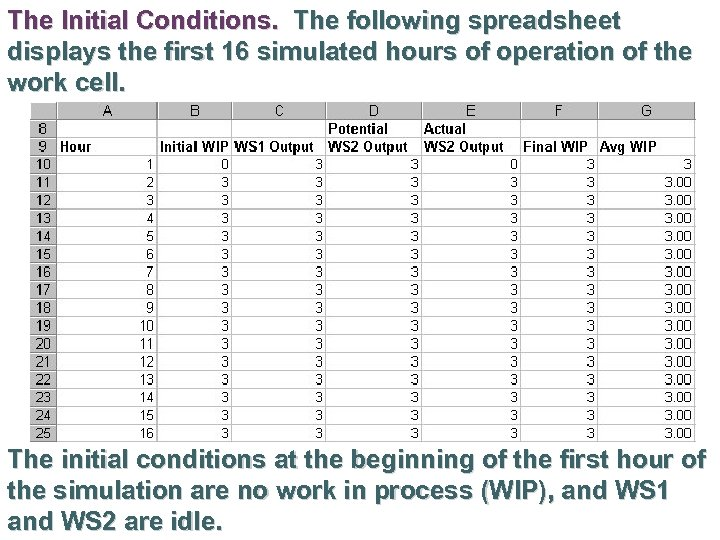The Initial Conditions. The following spreadsheet displays the first 16 simulated hours of operation of the work cell. The initial conditions at the beginning of the first hour of the simulation are no work in process (WIP), and WS 1 and WS 2 are idle.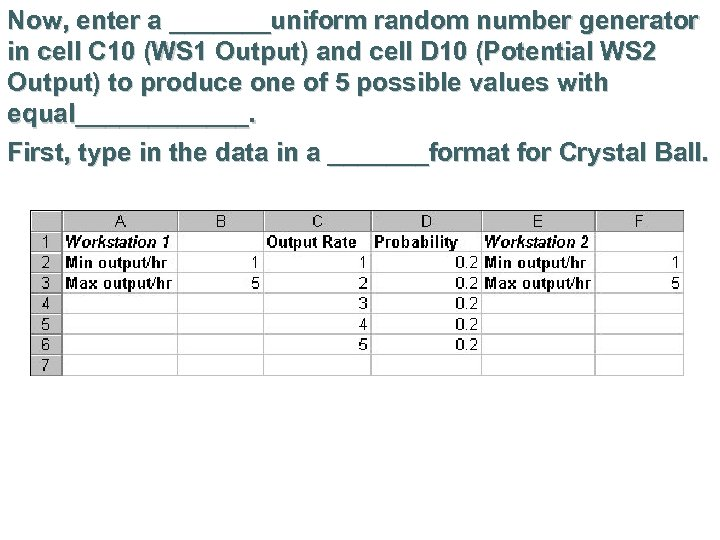Now, enter a _______uniform random number generator in cell C 10 (WS 1 Output) and cell D 10 (Potential WS 2 Output) to produce one of 5 possible values with equal______. First, type in the data in a _______format for Crystal Ball.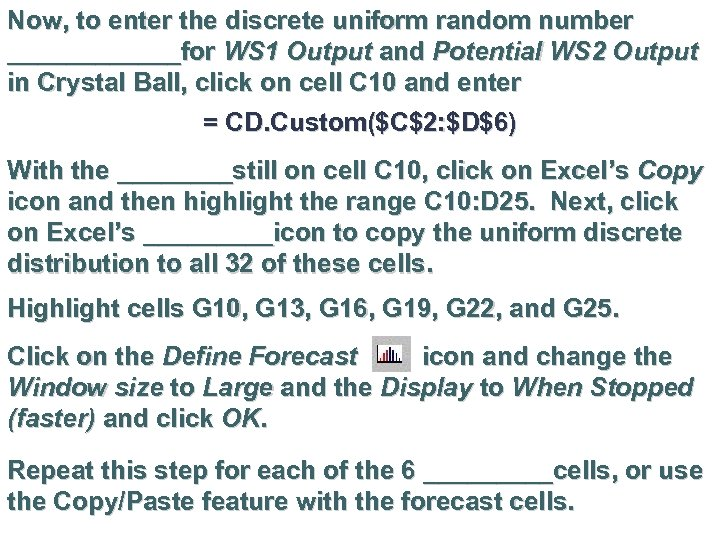Now, to enter the discrete uniform random number ______for WS 1 Output and Potential WS 2 Output in Crystal Ball, click on cell C 10 and enter = CD. Custom(\$C\$2: \$D\$6) With the ____still on cell C 10, click on Excel’s Copy icon and then highlight the range C 10: D 25. Next, click on Excel’s _____icon to copy the uniform discrete distribution to all 32 of these cells. Highlight cells G 10, G 13, G 16, G 19, G 22, and G 25. Click on the Define Forecast icon and change the Window size to Large and the Display to When Stopped (faster) and click OK. Repeat this step for each of the 6 _____cells, or use the Copy/Paste feature with the forecast cells.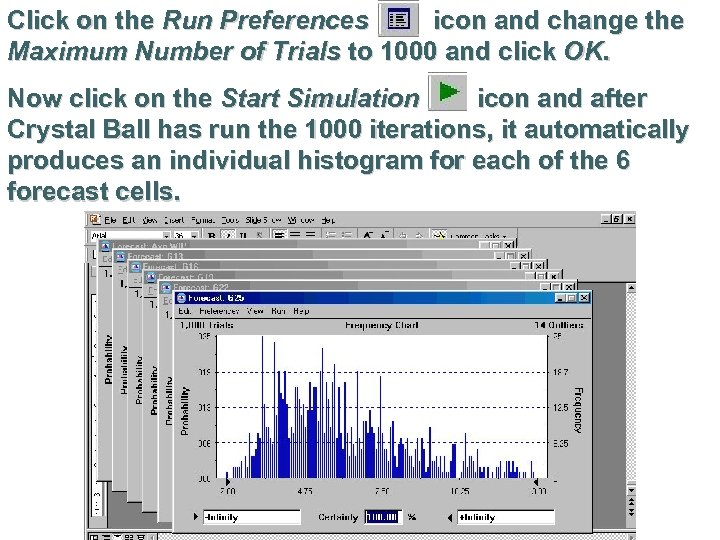Click on the Run Preferences icon and change the Maximum Number of Trials to 1000 and click OK. Now click on the Start Simulation icon and after Crystal Ball has run the 1000 iterations, it automatically produces an individual histogram for each of the 6 forecast cells.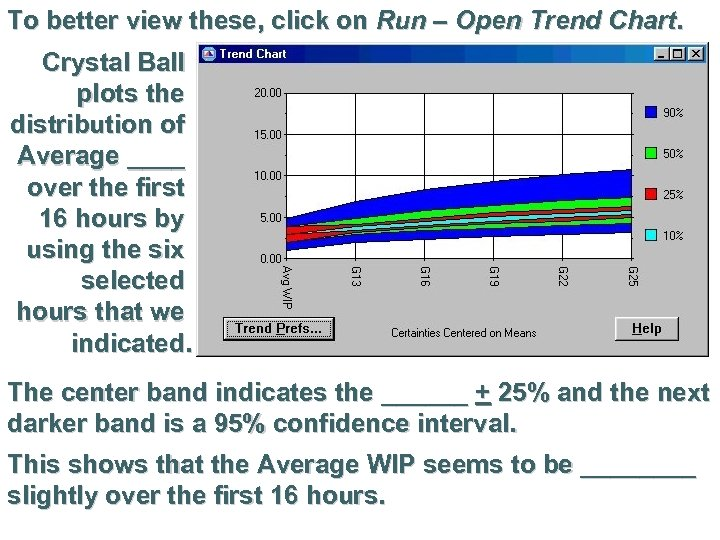To better view these, click on Run – Open Trend Chart. Crystal Ball plots the distribution of Average ____ over the first 16 hours by using the six selected hours that we indicated. The center band indicates the ______ + 25% and the next darker band is a 95% confidence interval. This shows that the Average WIP seems to be ____ slightly over the first 16 hours.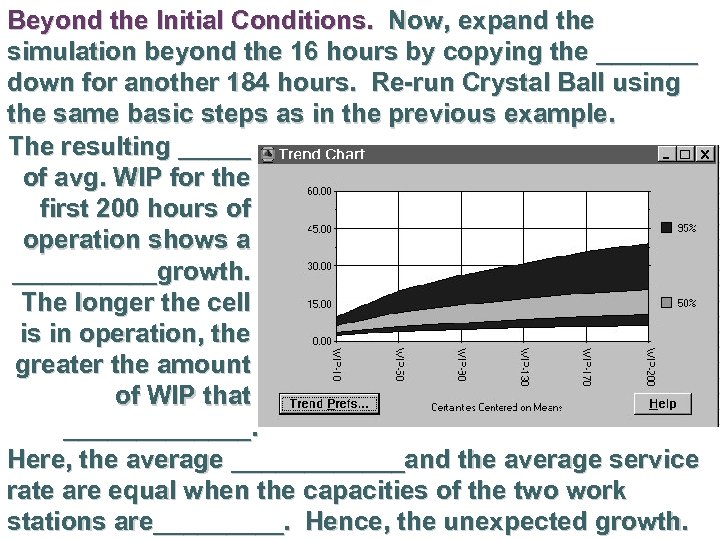Beyond the Initial Conditions. Now, expand the simulation beyond the 16 hours by copying the _______ down for another 184 hours. Re-run Crystal Ball using the same basic steps as in the previous example. The resulting _____ of avg. WIP for the first 200 hours of operation shows a _____growth. The longer the cell is in operation, the greater the amount of WIP that _______. Here, the average ______and the average service rate are equal when the capacities of the two work stations are_____. Hence, the unexpected growth.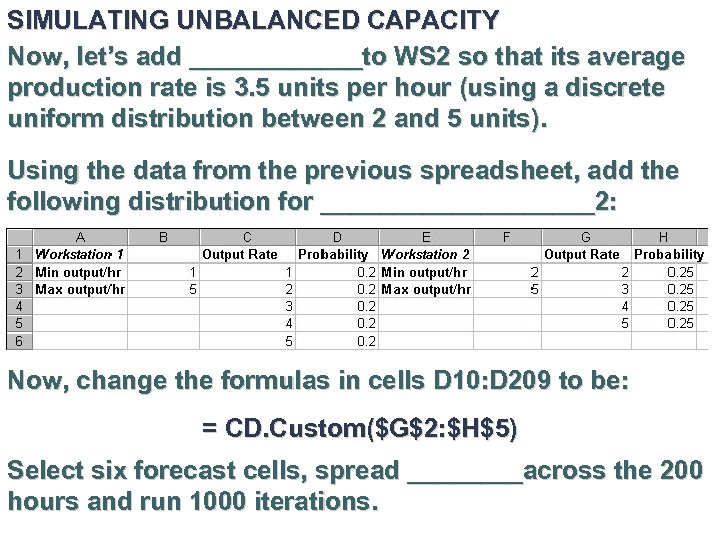SIMULATING UNBALANCED CAPACITY Now, let’s add ______to WS 2 so that its average production rate is 3. 5 units per hour (using a discrete uniform distribution between 2 and 5 units). Using the data from the previous spreadsheet, add the following distribution for __________2: Now, change the formulas in cells D 10: D 209 to be: = CD. Custom(\$G\$2: \$H\$5) Select six forecast cells, spread ____across the 200 hours and run 1000 iterations.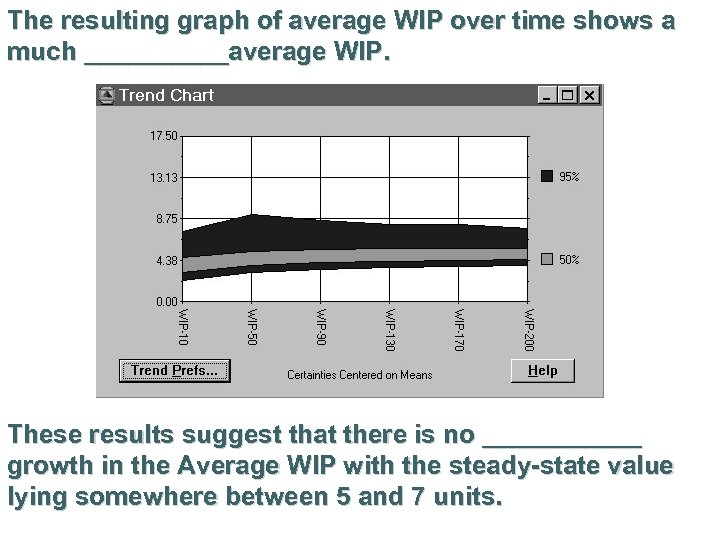The resulting graph of average WIP over time shows a much _____average WIP. These results suggest that there is no ______ growth in the Average WIP with the steady-state value lying somewhere between 5 and 7 units.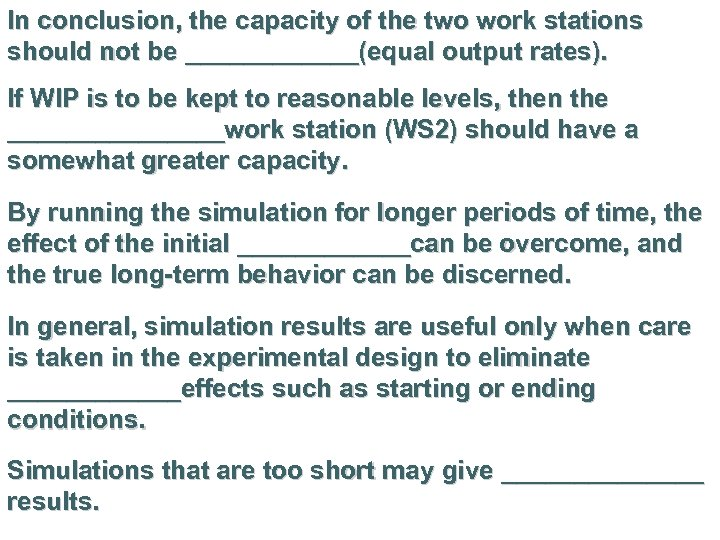In conclusion, the capacity of the two work stations should not be ______(equal output rates). If WIP is to be kept to reasonable levels, then the ________work station (WS 2) should have a somewhat greater capacity. By running the simulation for longer periods of time, the effect of the initial ______can be overcome, and the true long-term behavior can be discerned. In general, simulation results are useful only when care is taken in the experimental design to eliminate ______effects such as starting or ending conditions. Simulations that are too short may give _______ results.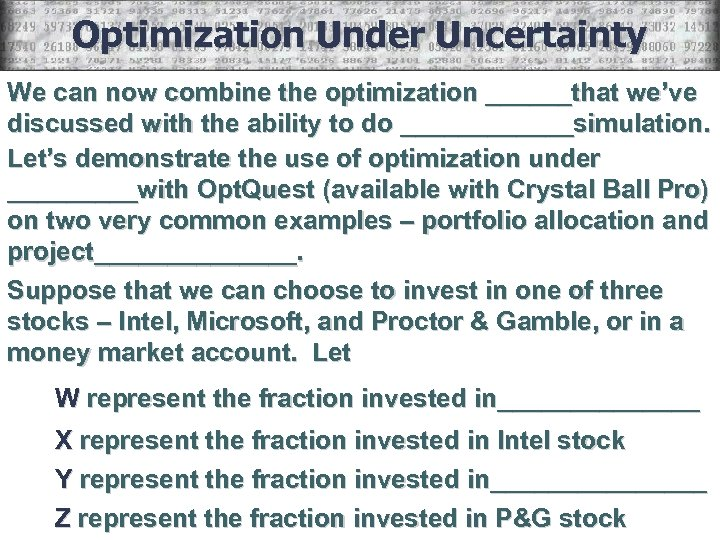Optimization Under Uncertainty We can now combine the optimization ______that we’ve discussed with the ability to do ______simulation. Let’s demonstrate the use of optimization under _____with Opt. Quest (available with Crystal Ball Pro) on two very common examples – portfolio allocation and project_______. Suppose that we can choose to invest in one of three stocks – Intel, Microsoft, and Proctor & Gamble, or in a money market account. Let W represent the fraction invested in_______ X represent the fraction invested in Intel stock Y represent the fraction invested in________ Z represent the fraction invested in P&G stock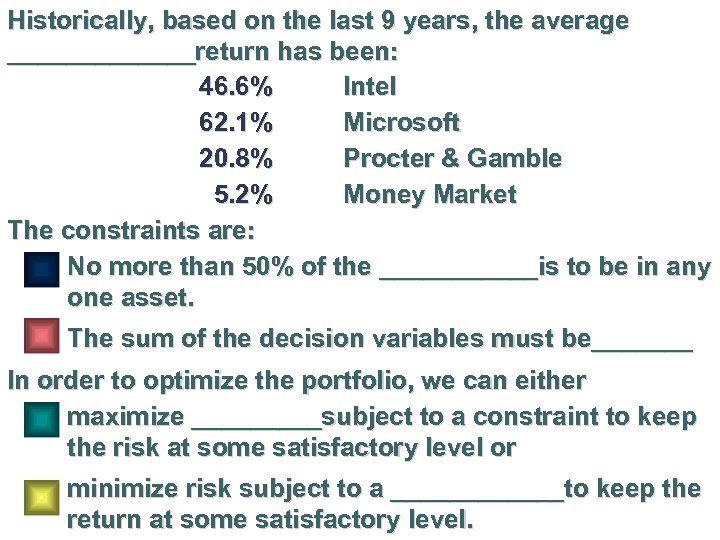Historically, based on the last 9 years, the average _______return has been: 46. 6% Intel 62. 1% Microsoft 20. 8% Procter & Gamble 5. 2% Money Market The constraints are: No more than 50% of the ______is to be in any one asset. The sum of the decision variables must be_______ In order to optimize the portfolio, we can either maximize _____subject to a constraint to keep the risk at some satisfactory level or minimize risk subject to a ______to keep the return at some satisfactory level.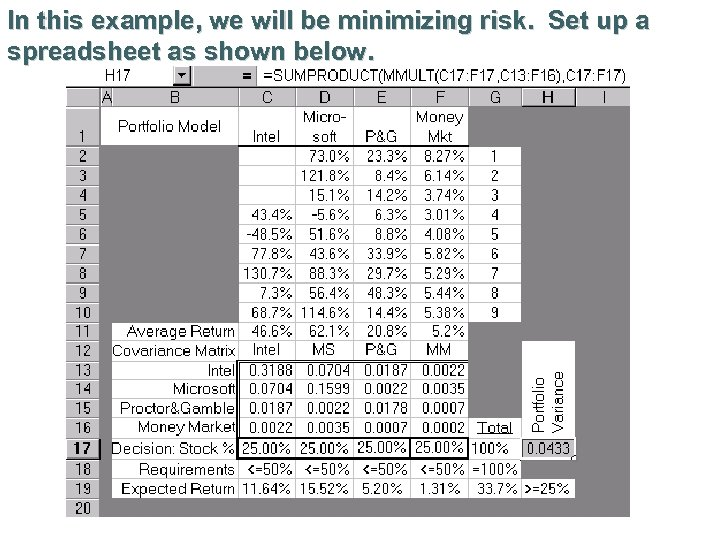In this example, we will be minimizing risk. Set up a spreadsheet as shown below.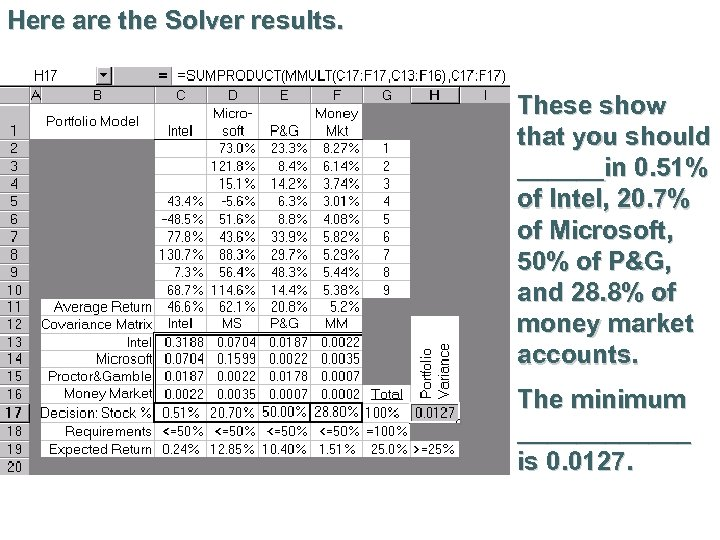Here are the Solver results. These show that you should ______in 0. 51% of Intel, 20. 7% of Microsoft, 50% of P&G, and 28. 8% of money market accounts. The minimum ______ is 0. 0127.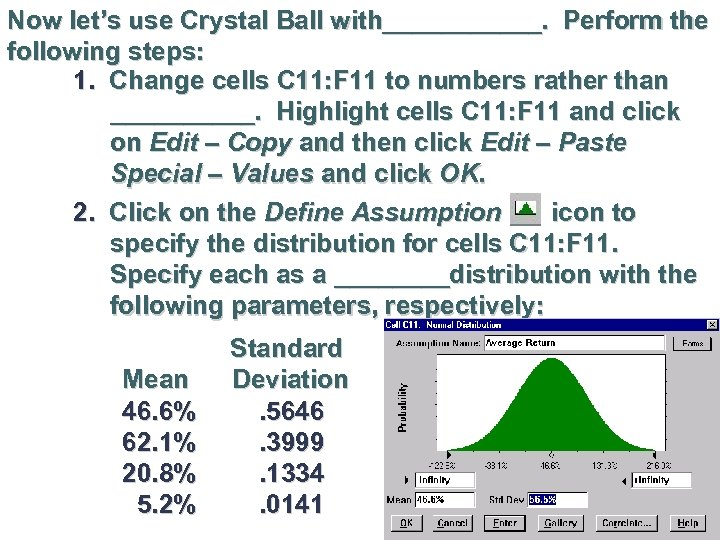Now let’s use Crystal Ball with______. Perform the following steps: 1. Change cells C 11: F 11 to numbers rather than _____. Highlight cells C 11: F 11 and click on Edit – Copy and then click Edit – Paste Special – Values and click OK. 2. Click on the Define Assumption icon to specify the distribution for cells C 11: F 11. Specify each as a ____distribution with the following parameters, respectively: Mean 46. 6% 62. 1% 20. 8% 5. 2% Standard Deviation. 5646. 3999. 1334. 0141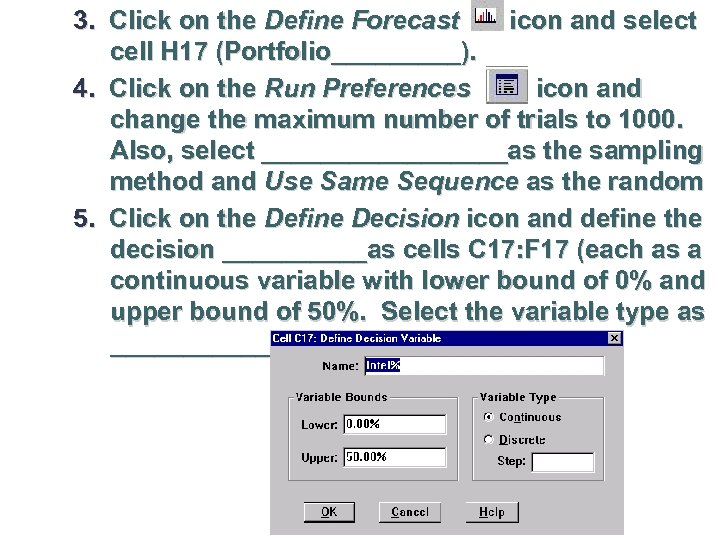3. Click on the Define Forecast icon and select cell H 17 (Portfolio_____). 4. Click on the Run Preferences icon and change the maximum number of trials to 1000. Also, select _________as the sampling method and Use Same Sequence as the random 5. Click on the Define Decision icon and define the decision _____as cells C 17: F 17 (each as a continuous variable with lower bound of 0% and upper bound of 50%. Select the variable type as ________.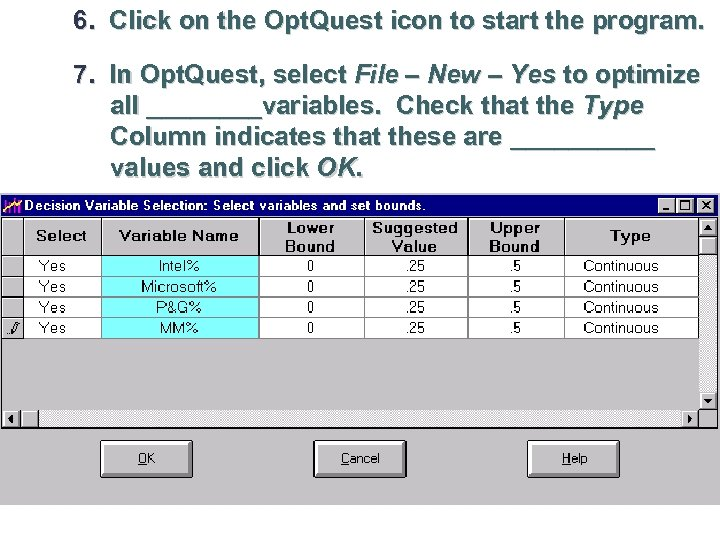6. Click on the Opt. Quest icon to start the program. 7. In Opt. Quest, select File – New – Yes to optimize all ____variables. Check that the Type Column indicates that these are _____ values and click OK.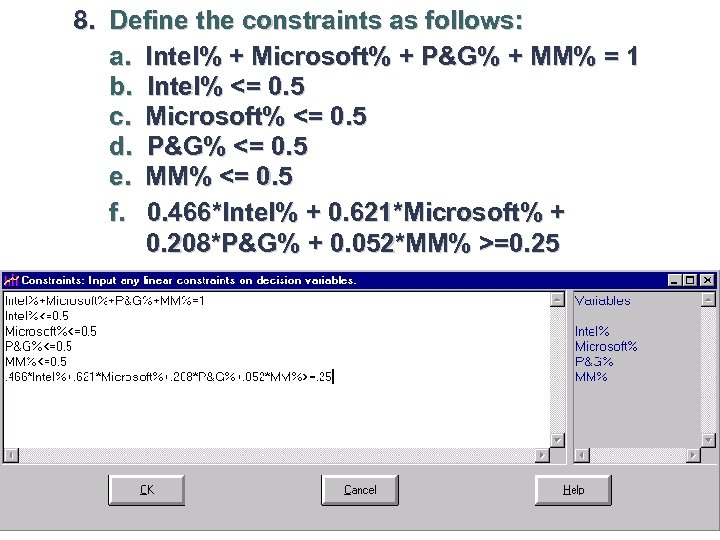8. Define the constraints as follows: a. Intel% + Microsoft% + P&G% + MM% = 1 b. Intel% <= 0. 5 c. Microsoft% <= 0. 5 d. P&G% <= 0. 5 e. MM% <= 0. 5 f. 0. 466*Intel% + 0. 621*Microsoft% + 0. 208*P&G% + 0. 052*MM% >=0. 25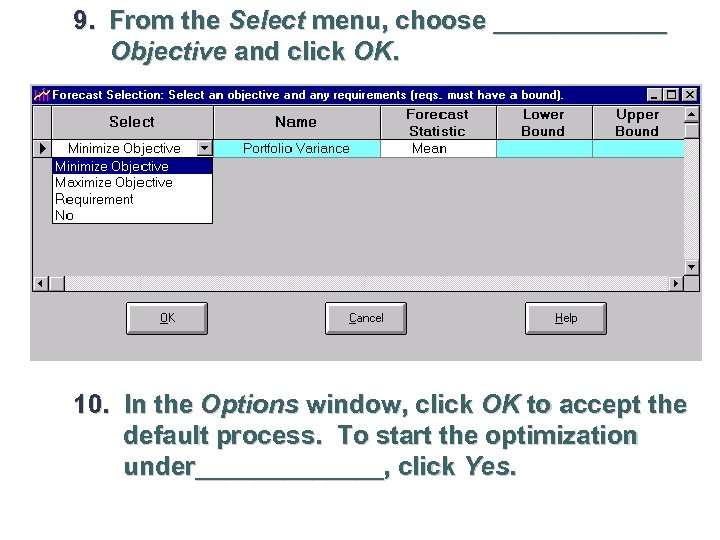9. From the Select menu, choose ______ Objective and click OK. 10. In the Options window, click OK to accept the default process. To start the optimization under_______, click Yes.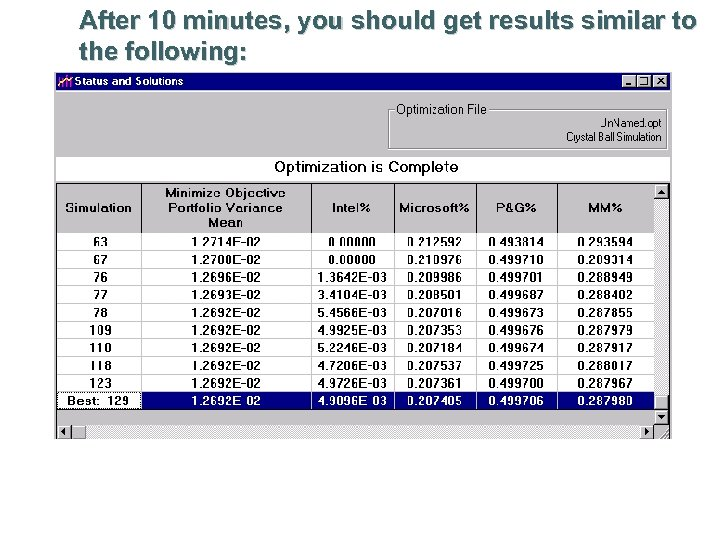After 10 minutes, you should get results similar to the following: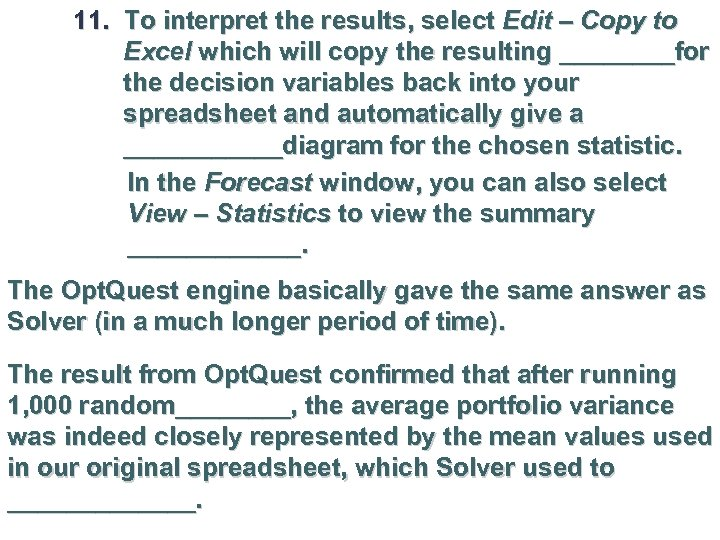11. To interpret the results, select Edit – Copy to Excel which will copy the resulting ____for the decision variables back into your spreadsheet and automatically give a ______diagram for the chosen statistic. In the Forecast window, you can also select View – Statistics to view the summary ______. The Opt. Quest engine basically gave the same answer as Solver (in a much longer period of time). The result from Opt. Quest confirmed that after running 1, 000 random____, the average portfolio variance was indeed closely represented by the mean values used in our original spreadsheet, which Solver used to _______.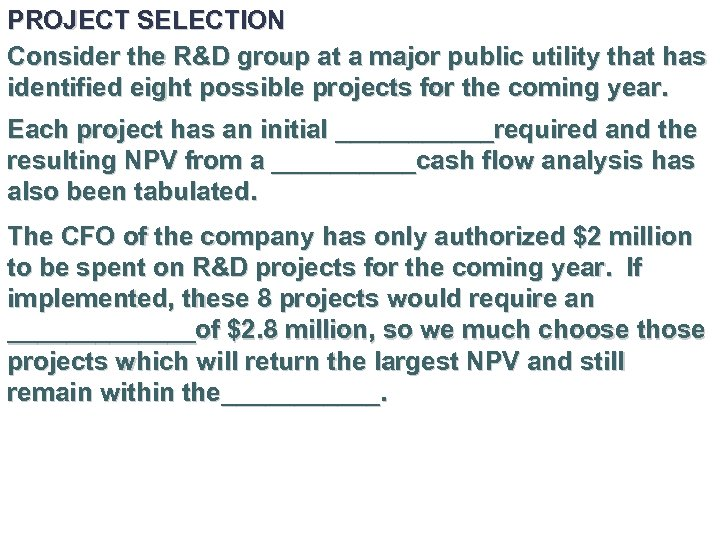PROJECT SELECTION Consider the R&D group at a major public utility that has identified eight possible projects for the coming year. Each project has an initial ______required and the resulting NPV from a _____cash flow analysis has also been tabulated. The CFO of the company has only authorized \$2 million to be spent on R&D projects for the coming year. If implemented, these 8 projects would require an _______of \$2. 8 million, so we much choose those projects which will return the largest NPV and still remain within the______.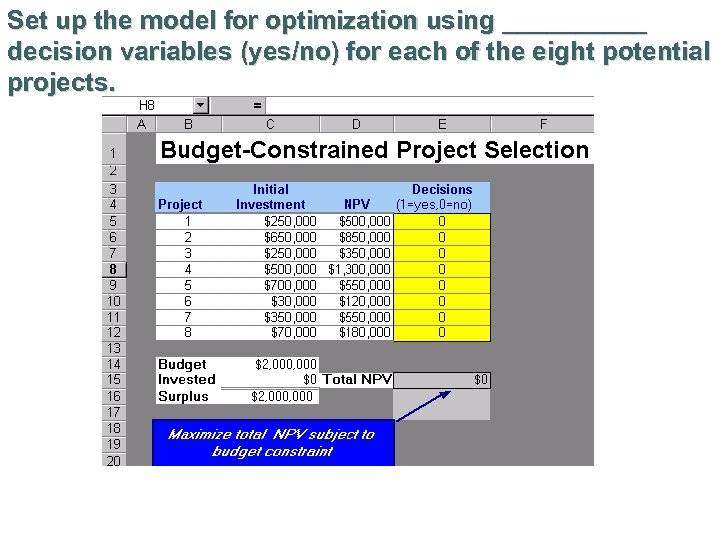Set up the model for optimization using _____ decision variables (yes/no) for each of the eight potential projects.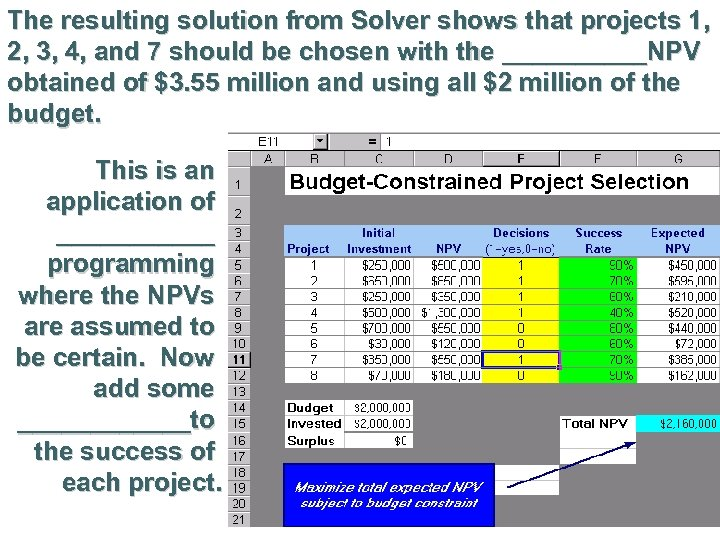The resulting solution from Solver shows that projects 1, 2, 3, 4, and 7 should be chosen with the _____NPV obtained of \$3. 55 million and using all \$2 million of the budget. This is an application of ______ programming where the NPVs are assumed to be certain. Now add some ______to the success of each project.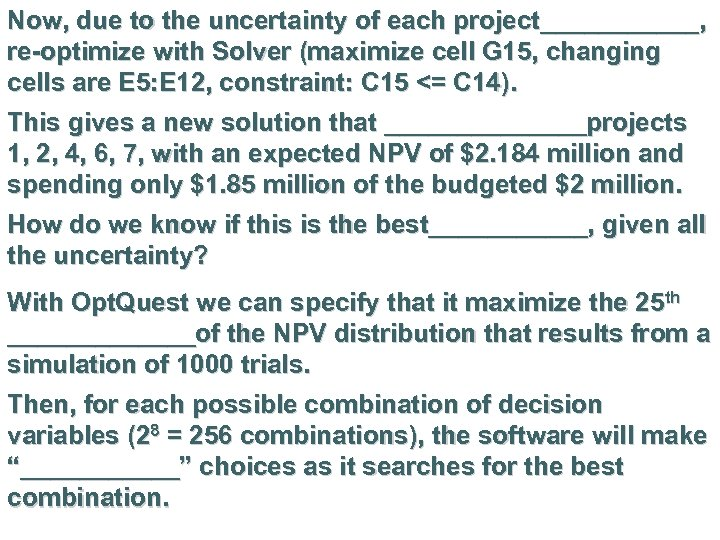Now, due to the uncertainty of each project______, re-optimize with Solver (maximize cell G 15, changing cells are E 5: E 12, constraint: C 15 <= C 14). This gives a new solution that _______projects 1, 2, 4, 6, 7, with an expected NPV of \$2. 184 million and spending only \$1. 85 million of the budgeted \$2 million. How do we know if this is the best______, given all the uncertainty? With Opt. Quest we can specify that it maximize the 25 th _______of the NPV distribution that results from a simulation of 1000 trials. Then, for each possible combination of decision variables (28 = 256 combinations), the software will make “______” choices as it searches for the best combination.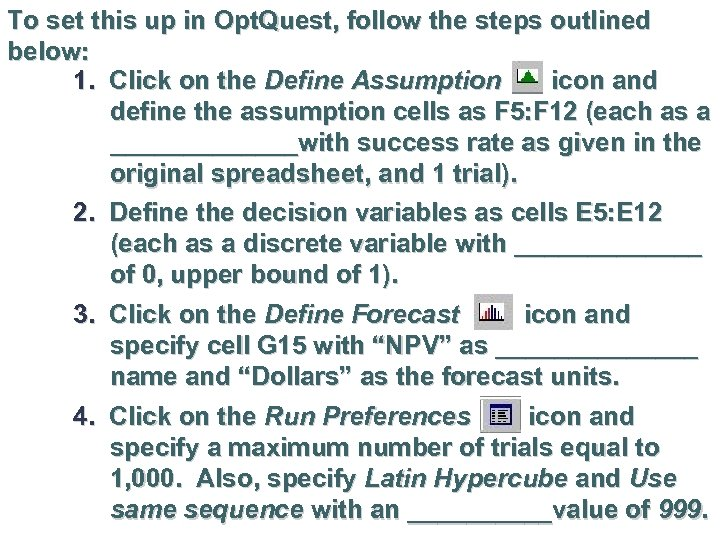To set this up in Opt. Quest, follow the steps outlined below: 1. Click on the Define Assumption icon and define the assumption cells as F 5: F 12 (each as a _______with success rate as given in the original spreadsheet, and 1 trial). 2. Define the decision variables as cells E 5: E 12 (each as a discrete variable with _______ of 0, upper bound of 1). 3. Click on the Define Forecast icon and specify cell G 15 with “NPV” as _______ name and “Dollars” as the forecast units. 4. Click on the Run Preferences icon and specify a maximum number of trials equal to 1, 000. Also, specify Latin Hypercube and Use same sequence with an _____value of 999.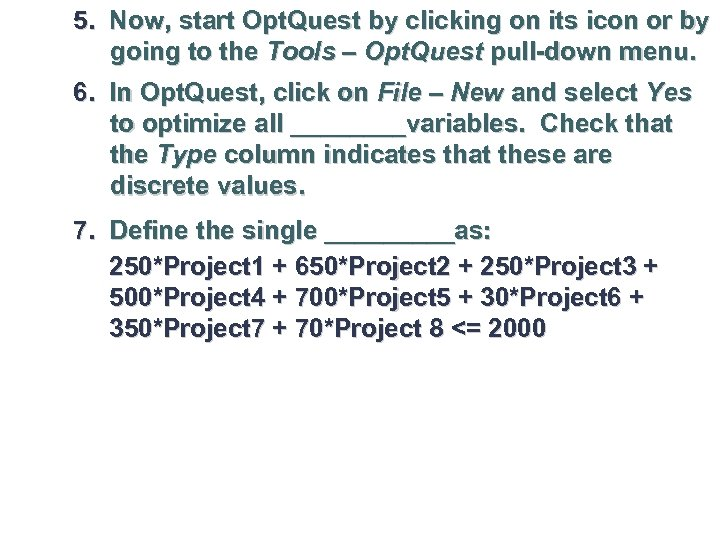5. Now, start Opt. Quest by clicking on its icon or by going to the Tools – Opt. Quest pull-down menu. 6. In Opt. Quest, click on File – New and select Yes to optimize all ____variables. Check that the Type column indicates that these are discrete values. 7. Define the single _____as: 250*Project 1 + 650*Project 2 + 250*Project 3 + 500*Project 4 + 700*Project 5 + 30*Project 6 + 350*Project 7 + 70*Project 8 <= 2000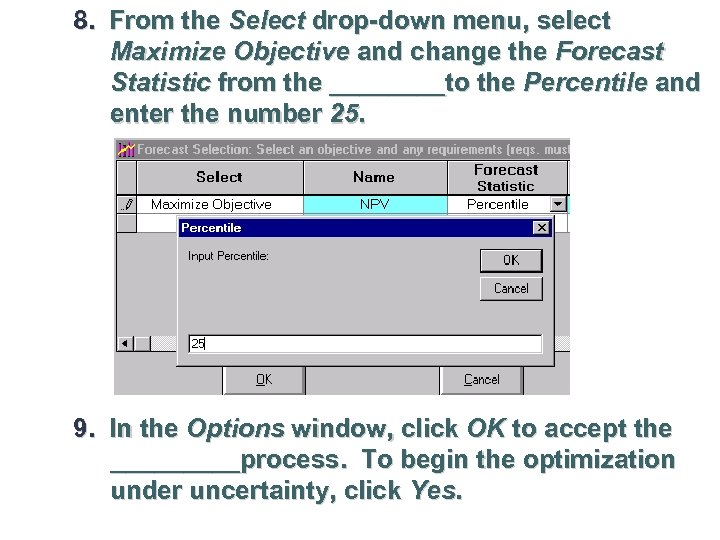8. From the Select drop-down menu, select Maximize Objective and change the Forecast Statistic from the ____to the Percentile and enter the number 25. 9. In the Options window, click OK to accept the _____process. To begin the optimization under uncertainty, click Yes.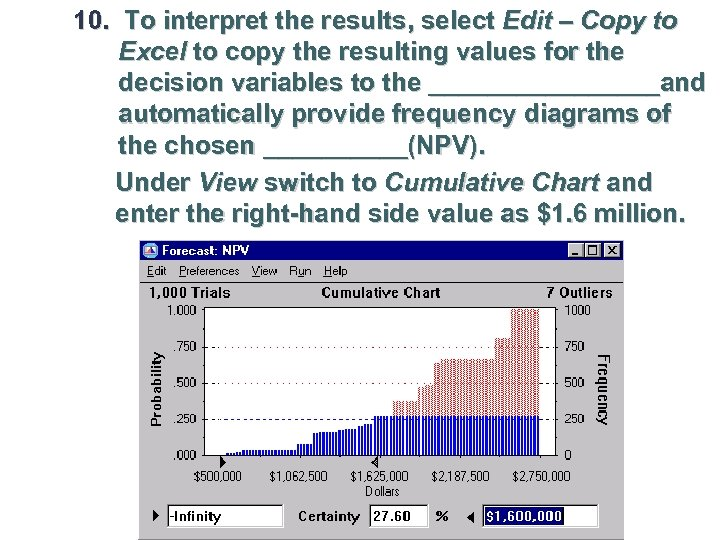10. To interpret the results, select Edit – Copy to Excel to copy the resulting values for the decision variables to the ________and automatically provide frequency diagrams of the chosen _____(NPV). Under View switch to Cumulative Chart and enter the right-hand side value as \$1. 6 million.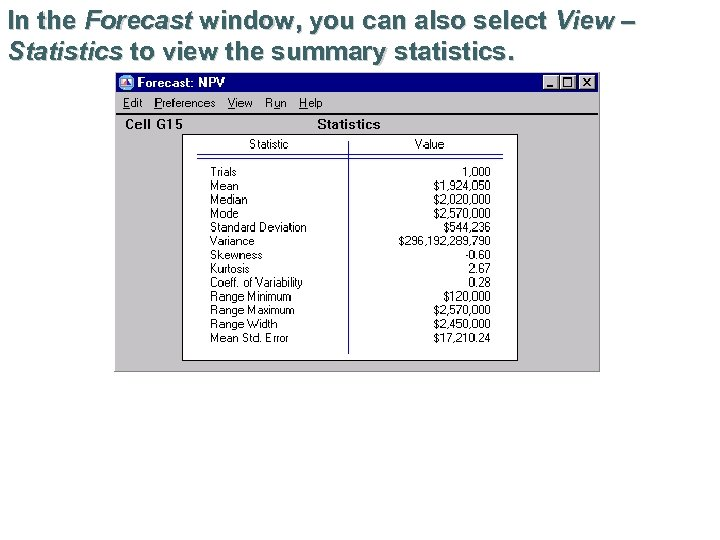In the Forecast window, you can also select View – Statistics to view the summary statistics.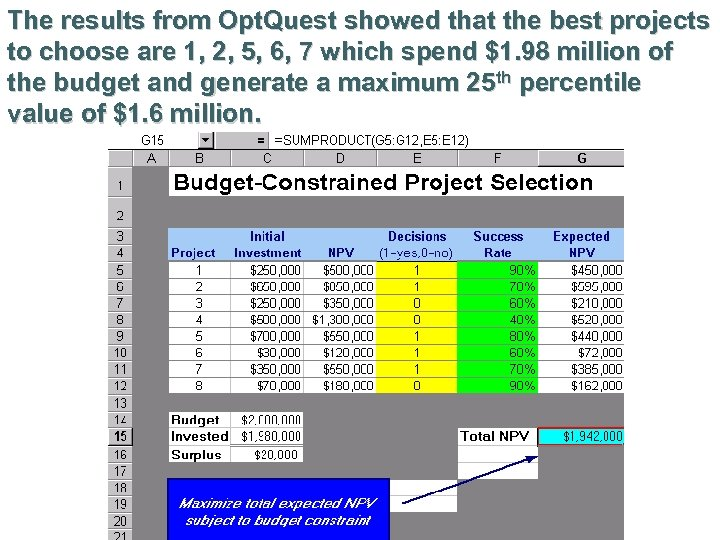The results from Opt. Quest showed that the best projects to choose are 1, 2, 5, 6, 7 which spend \$1. 98 million of the budget and generate a maximum 25 th percentile value of \$1. 6 million.